This vignette can be referred to by citing the package:

citation("see")
#>
#> To cite package 'see' in publications use:
#>
#>   Lüdecke et al., (2021). see: An R Package for Visualizing Statistical
#>   Models. Journal of Open Source Software, 6(64), 3393.
#>   https://doi.org/10.21105/joss.03393
#>
#> A BibTeX entry for LaTeX users is
#>
#>   @Article{,
#>     title = {{see}: An {R} Package for Visualizing Statistical Models},
#>     author = {Daniel Lüdecke and Indrajeet Patil and Mattan S. Ben-Shachar and Brenton M. Wiernik and Philip Waggoner and Dominique Makowski},
#>     journal = {Journal of Open Source Software},
#>     year = {2021},
#>     volume = {6},
#>     number = {64},
#>     pages = {3393},
#>     doi = {10.21105/joss.03393},
#>   }

## Introduction

parameters’ primary goal is to provide utilities for processing the parameters of various statistical models (see here for a list of supported models). Beyond computing p-values, CIs, Bayesian indices and other measures for a wide variety of models, this package implements features like bootstrapping of parameters and models, feature reduction (feature extraction and variable selection), or tools for data reduction like functions to perform cluster, factor or principal component analysis.

Another important goal of the parameters package is to facilitate and streamline the process of reporting results of statistical models, which includes the easy and intuitive calculation of standardized estimates or robust standard errors and p-values. parameters therefor offers a simple and unified syntax to process a large variety of (model) objects from many different packages.

For more, see: https://easystats.github.io/parameters/

## Setup and Model Fitting

library(parameters)
library(effectsize)
library(insight)
library(see)
library(glmmTMB)
library(lme4)
library(lavaan)
library(metafor)
library(ggplot2)
library(splines)
data("Salamanders")
data("iris")
data("sleepstudy")
data("qol_cancer")

set.seed(12345)
sleepstudy$grp <- sample(1:5, size = 180, replace = TRUE) theme_set(theme_modern()) # fit three example model model1 <- glmmTMB( count ~ spp + mined + (1 | site), ziformula = ~mined, family = poisson(), data = Salamanders ) model_parameters(model1, effects = "fixed") #> # Fixed Effects #> #> Parameter | Log-Mean | SE | 95% CI | z | p #> --------------------------------------------------------------- #> (Intercept) | -0.36 | 0.28 | [-0.90, 0.18] | -1.30 | 0.194 #> spp [PR] | -1.27 | 0.24 | [-1.74, -0.80] | -5.27 | < .001 #> spp [DM] | 0.27 | 0.14 | [ 0.00, 0.54] | 1.95 | 0.051 #> spp [EC-A] | -0.57 | 0.21 | [-0.97, -0.16] | -2.75 | 0.006 #> spp [EC-L] | 0.67 | 0.13 | [ 0.41, 0.92] | 5.20 | < .001 #> spp [DES-L] | 0.63 | 0.13 | [ 0.38, 0.87] | 4.96 | < .001 #> spp [DF] | 0.12 | 0.15 | [-0.17, 0.40] | 0.78 | 0.435 #> mined [no] | 1.27 | 0.27 | [ 0.74, 1.80] | 4.72 | < .001 #> #> # Zero-Inflated #> #> Parameter | Log-Odds | SE | 95% CI | z | p #> --------------------------------------------------------------- #> (Intercept) | 0.79 | 0.27 | [ 0.26, 1.32] | 2.90 | 0.004 #> mined [no] | -1.84 | 0.31 | [-2.46, -1.23] | -5.87 | < .001 model2 <- lm(Sepal.Length ~ Species * bs(Petal.Width, degree = 2), data = iris) model_parameters(model2, effects = "fixed") #> Parameter | Coefficient | SE | 95% CI | t(141) | p #> --------------------------------------------------------------------------------------------------------- #> (Intercept) | 4.79 | 0.17 | [ 4.45, 5.13] | 27.66 | < .001 #> Species [versicolor] | -3.73 | 2.14 | [ -7.96, 0.50] | -1.74 | 0.083 #> Species [virginica] | -2.67 | 2.88 | [ -8.36, 3.03] | -0.93 | 0.356 #> Petal Width [1st degree] | 2.53 | 2.36 | [ -2.13, 7.20] | 1.07 | 0.285 #> Petal Width [2nd degree] | -11.18 | 21.14 | [-52.98, 30.62] | -0.53 | 0.598 #> Species [versicolor] * Petal Width [1st degree] | 5.48 | 4.84 | [ -4.09, 15.05] | 1.13 | 0.260 #> Species [virginica] * Petal Width [1st degree] | 2.37 | 4.35 | [ -6.22, 10.96] | 0.54 | 0.587 #> Species [versicolor] * Petal Width [2nd degree] | 14.84 | 21.16 | [-26.99, 56.68] | 0.70 | 0.484 #> Species [virginica] * Petal Width [2nd degree] | 15.81 | 21.32 | [-26.35, 57.96] | 0.74 | 0.460 model3 <- lmer( Reaction ~ Days + (1 | grp) + (1 | Subject), data = sleepstudy ) model4 <- lm(QoL ~ time + age + education, data = qol_cancer) ## Model Parameters (related function documentation) The plot()-method for model_parameters() creates a so called “forest plot”. In case of models with multiple components, parameters are separated into facets by model component. result <- model_parameters(model1, effects = "fixed") plot(result)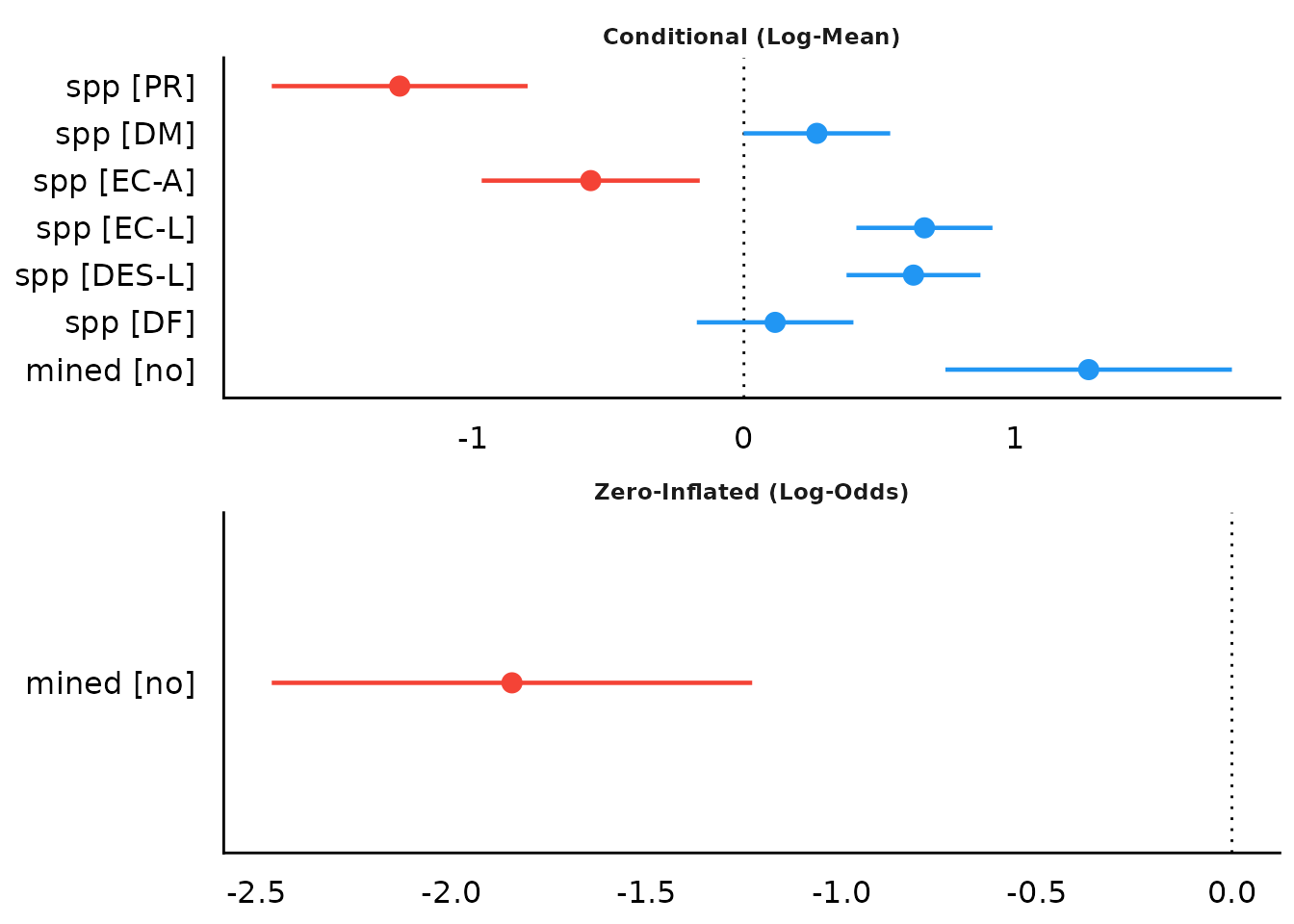When show_labels is TRUE, coefficients and confidence intervals are added to the plot. Adjust the text size with size_text. plot(result, show_labels = TRUE, size_text = 4)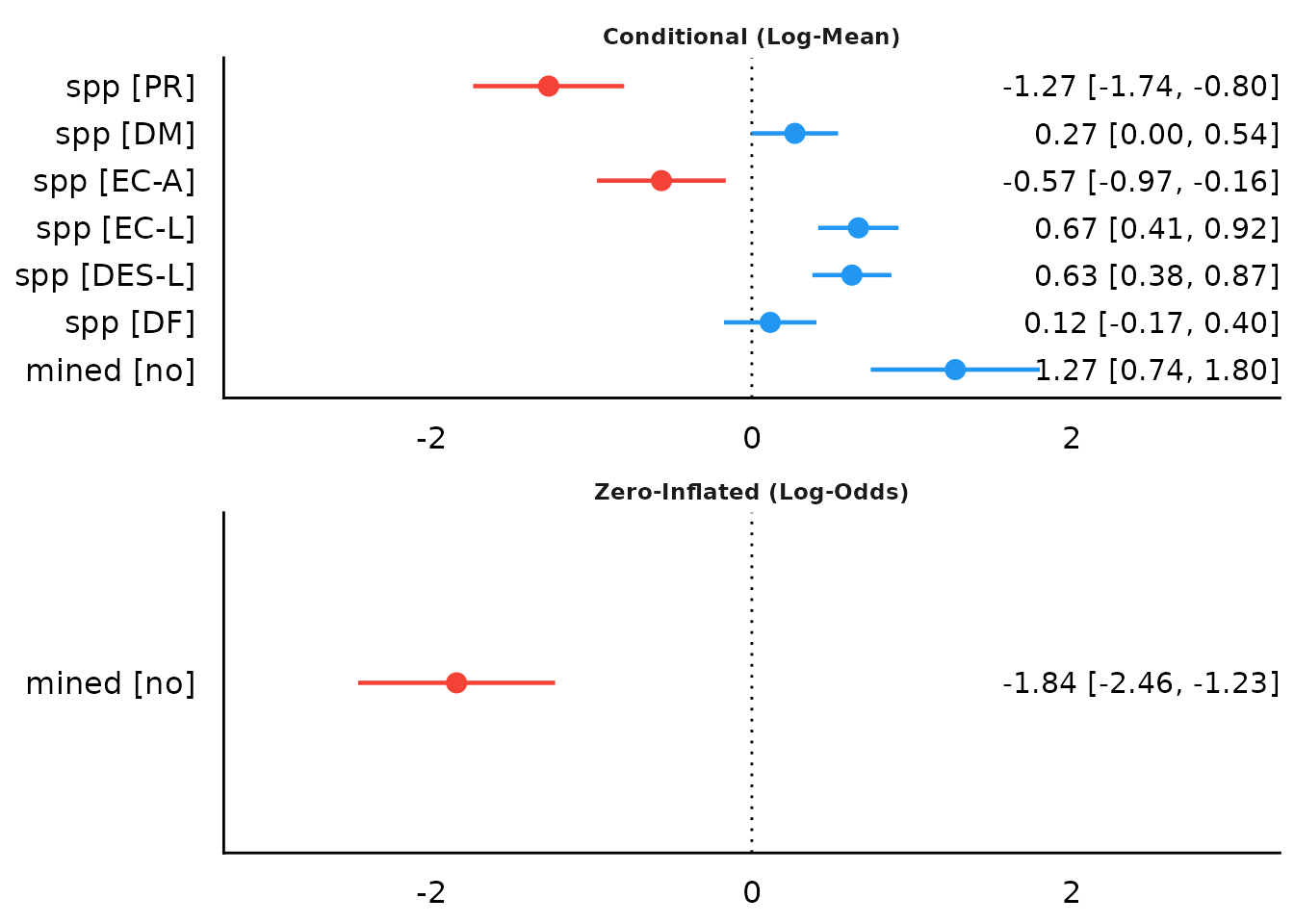This also works for exponentiated coefficients. result <- model_parameters(model1, exponentiate = TRUE, effects = "fixed") plot(result, show_labels = TRUE, size_text = 4)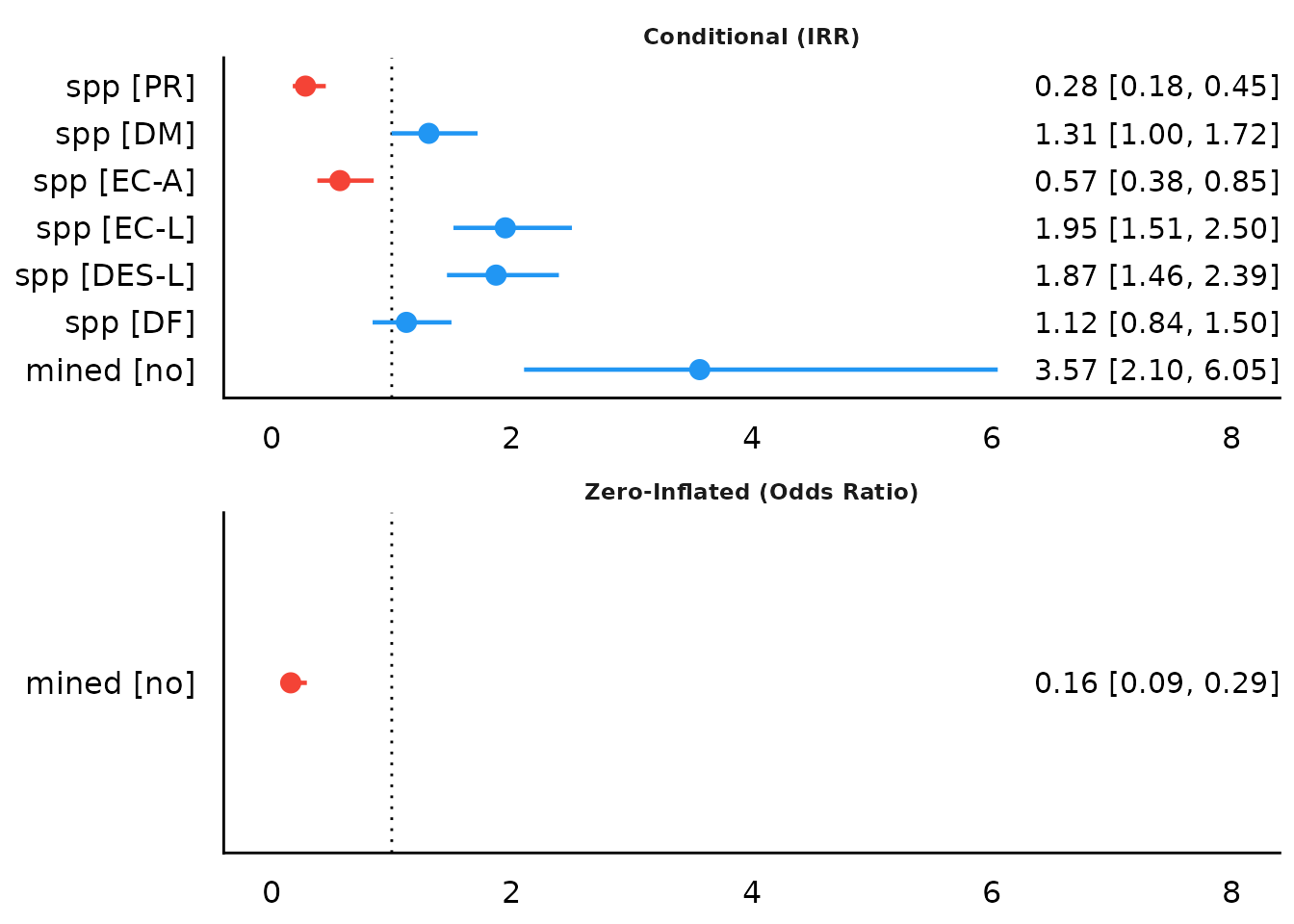It is also possible to plot only the count-model component. This is done in model_parameters() via the component argument. In easystats-functions, the count-component has the more generic name "conditional". result <- model_parameters(model1, exponentiate = TRUE, component = "conditional") plot(result)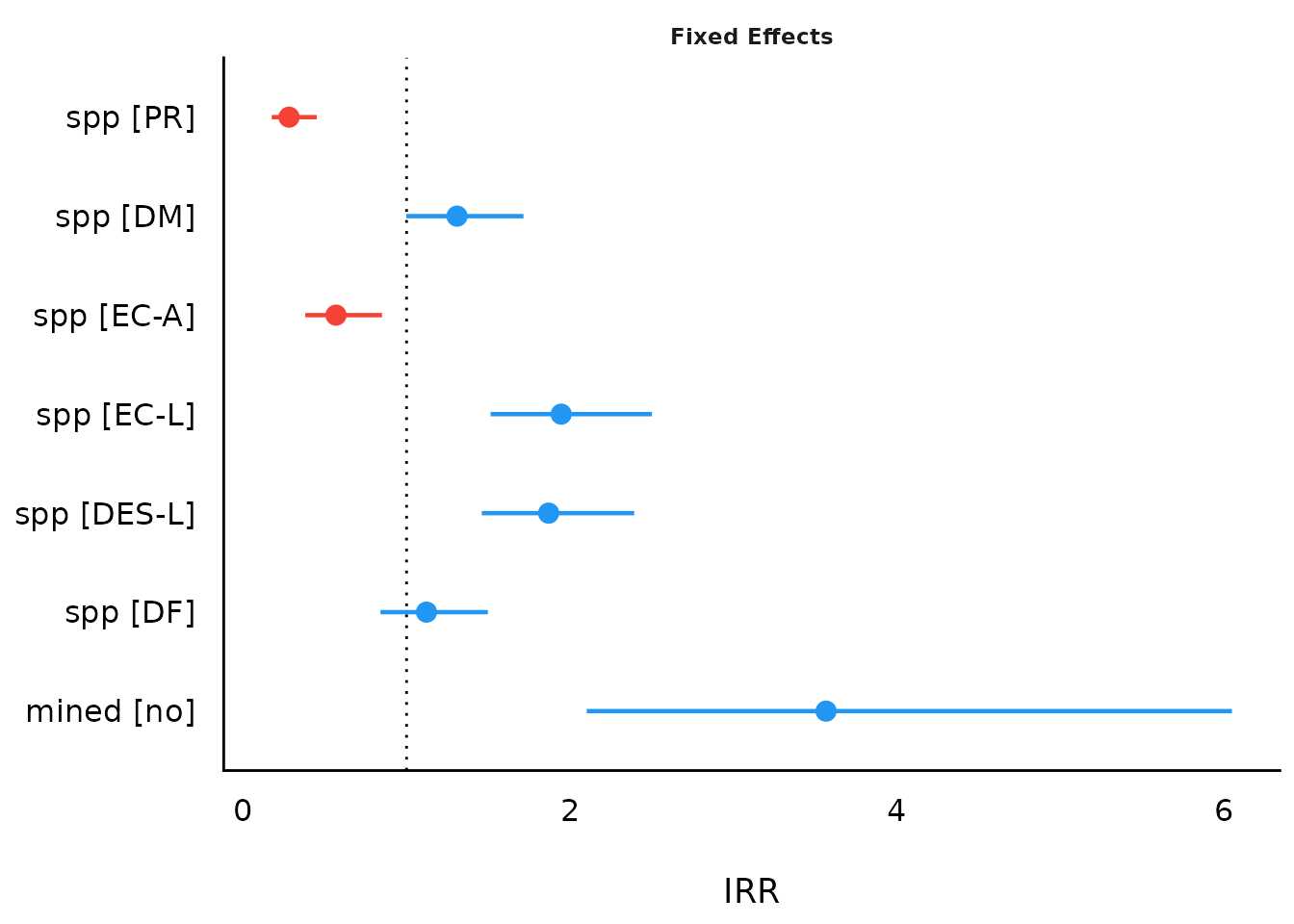As compared to the classical summary()-output, model_parameters(), and hence the plot()-method, tries to create human readable, prettier parameters names. result <- model_parameters(model2) plot(result)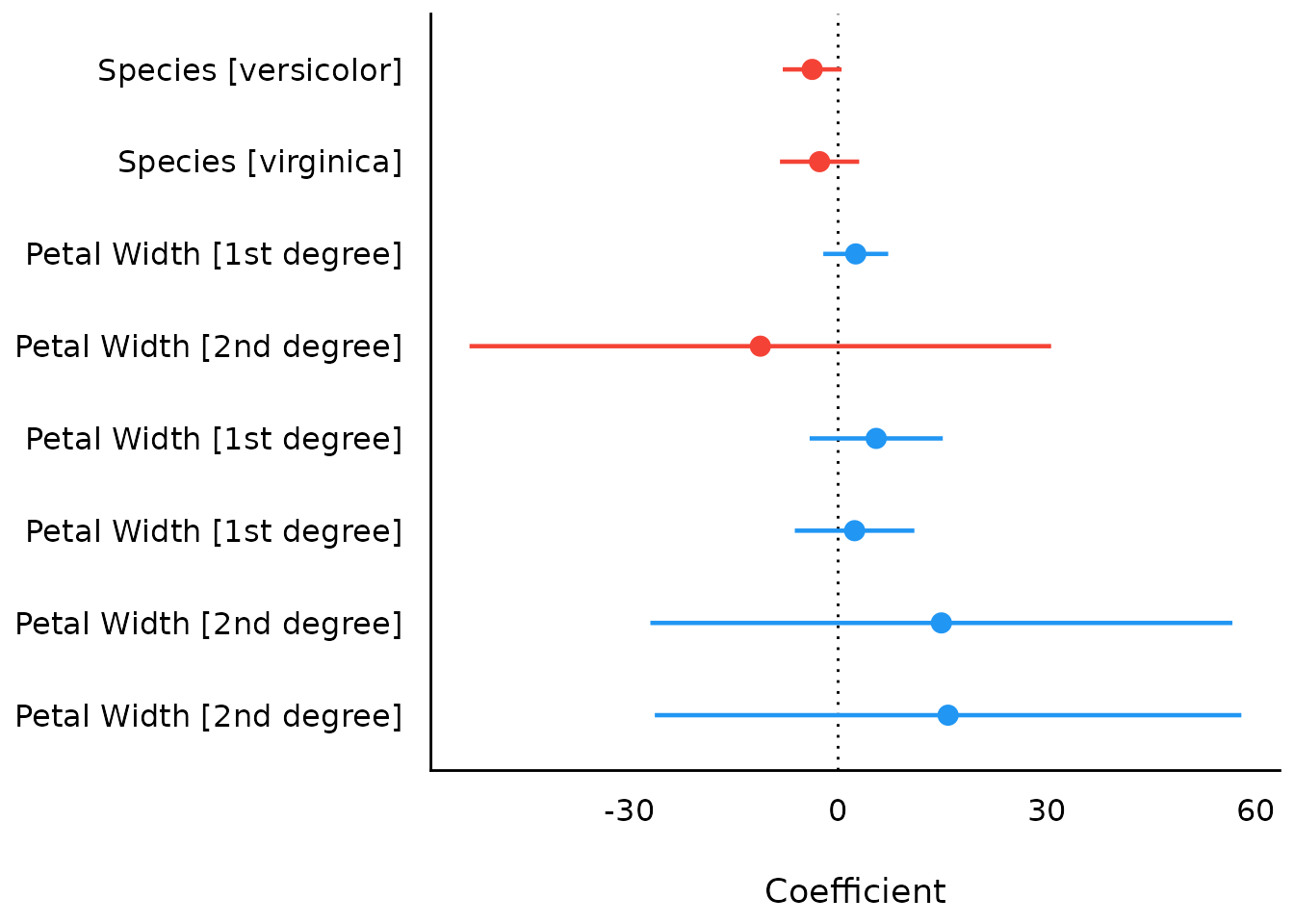### Changing parameter names in the plot By default, model_parameters() returns a data frame, where the parameter names are found in the column Parameter. These names are used by default in the generated plot: plot(model_parameters(model4))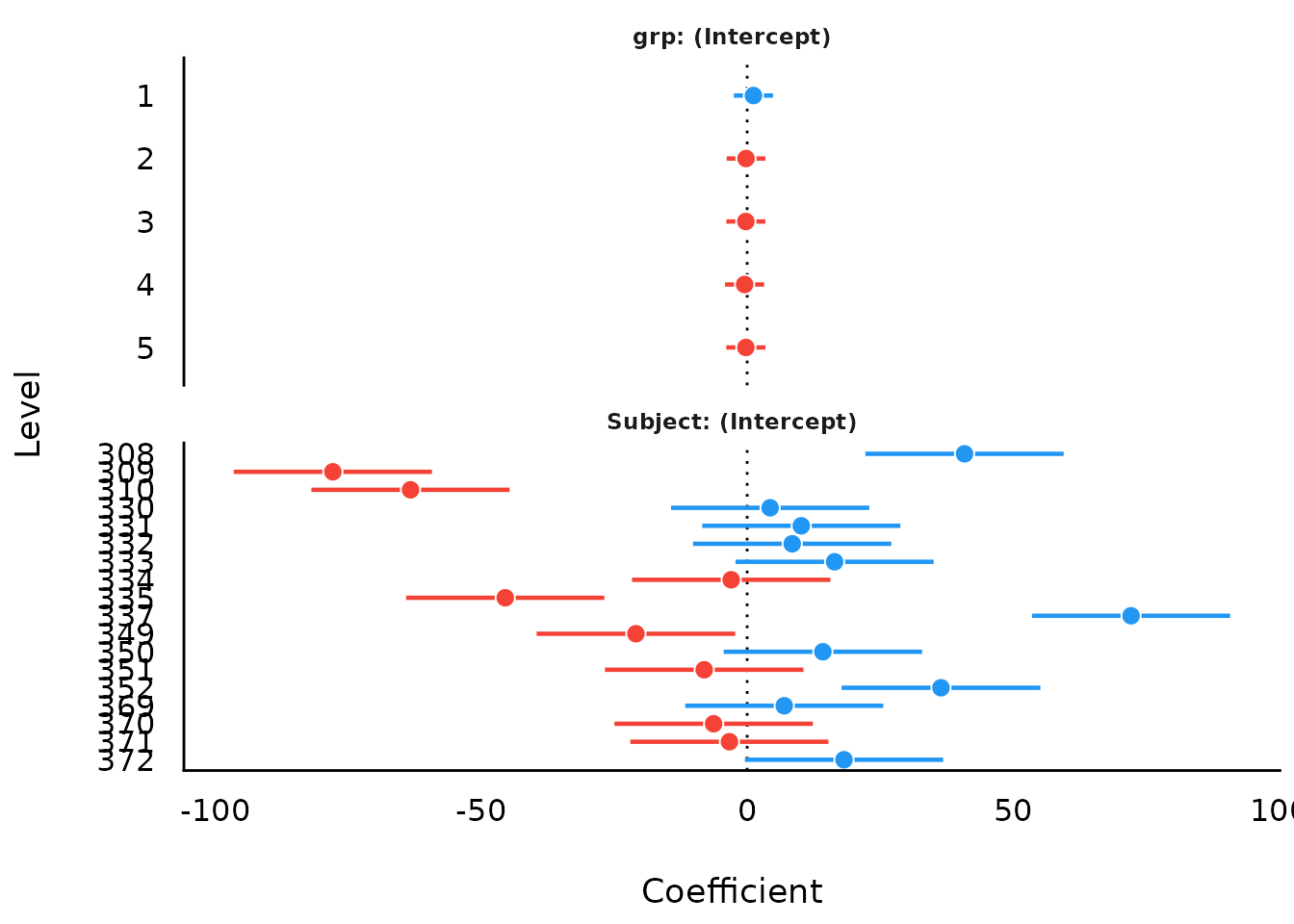However, there are several ways to change the the names of the parameters. Since plot() returns ggplot objects, these can be easily modified, e.g. by adding a scale_y_discrete() layer. library(ggplot2) plot(model_parameters(model4)) + scale_y_discrete(labels = c("Time", "Age", "Education (mid-level)", "Eucation (high-level)"))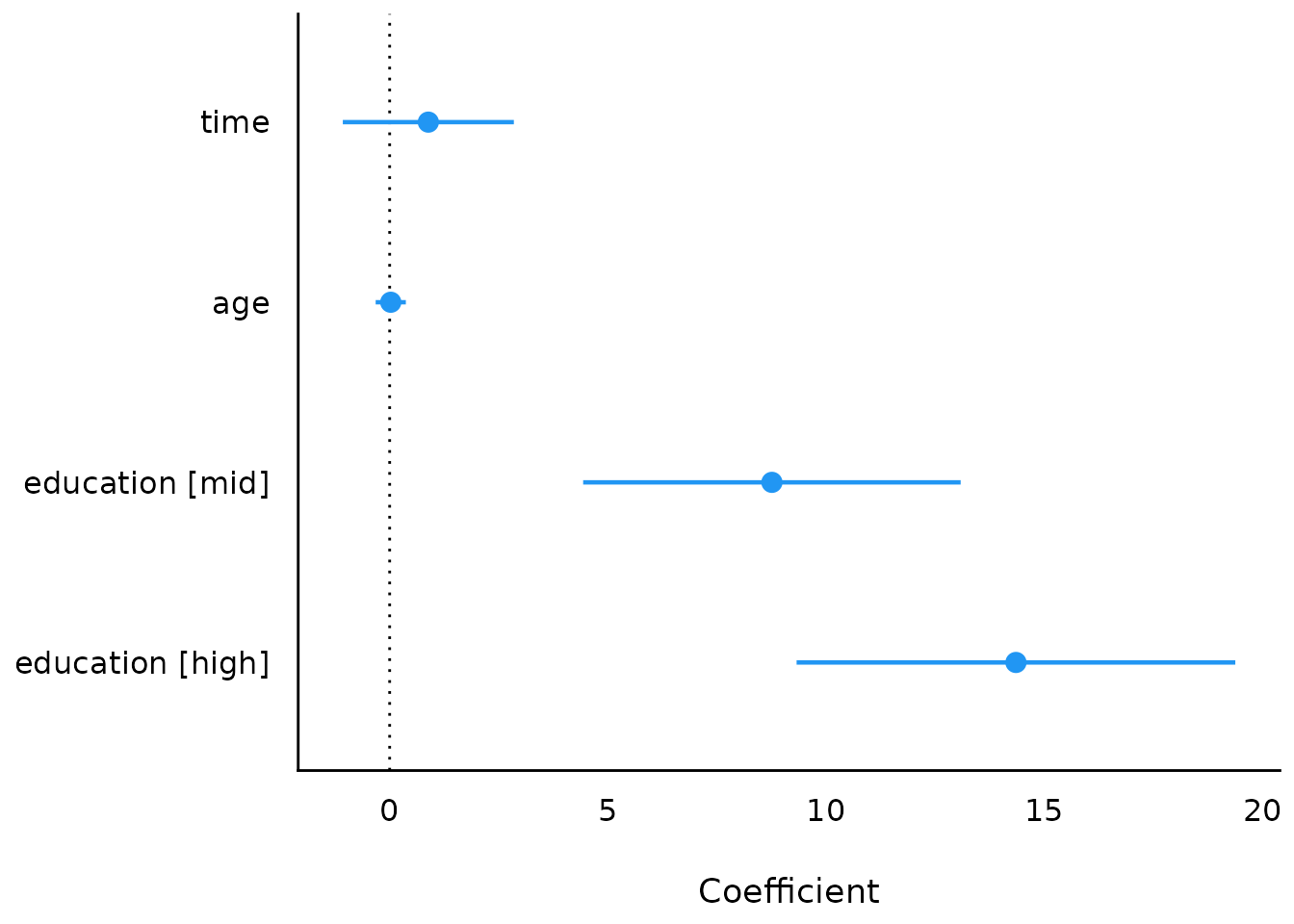Another way would be changing the values of the Parameter column before calling plot(): mp <- model_parameters(model4) mp$Parameter <- c("(Intercept)", "Time", "Age", "Education (mid-level)",
"Eucation (high-level)")
plot(mp)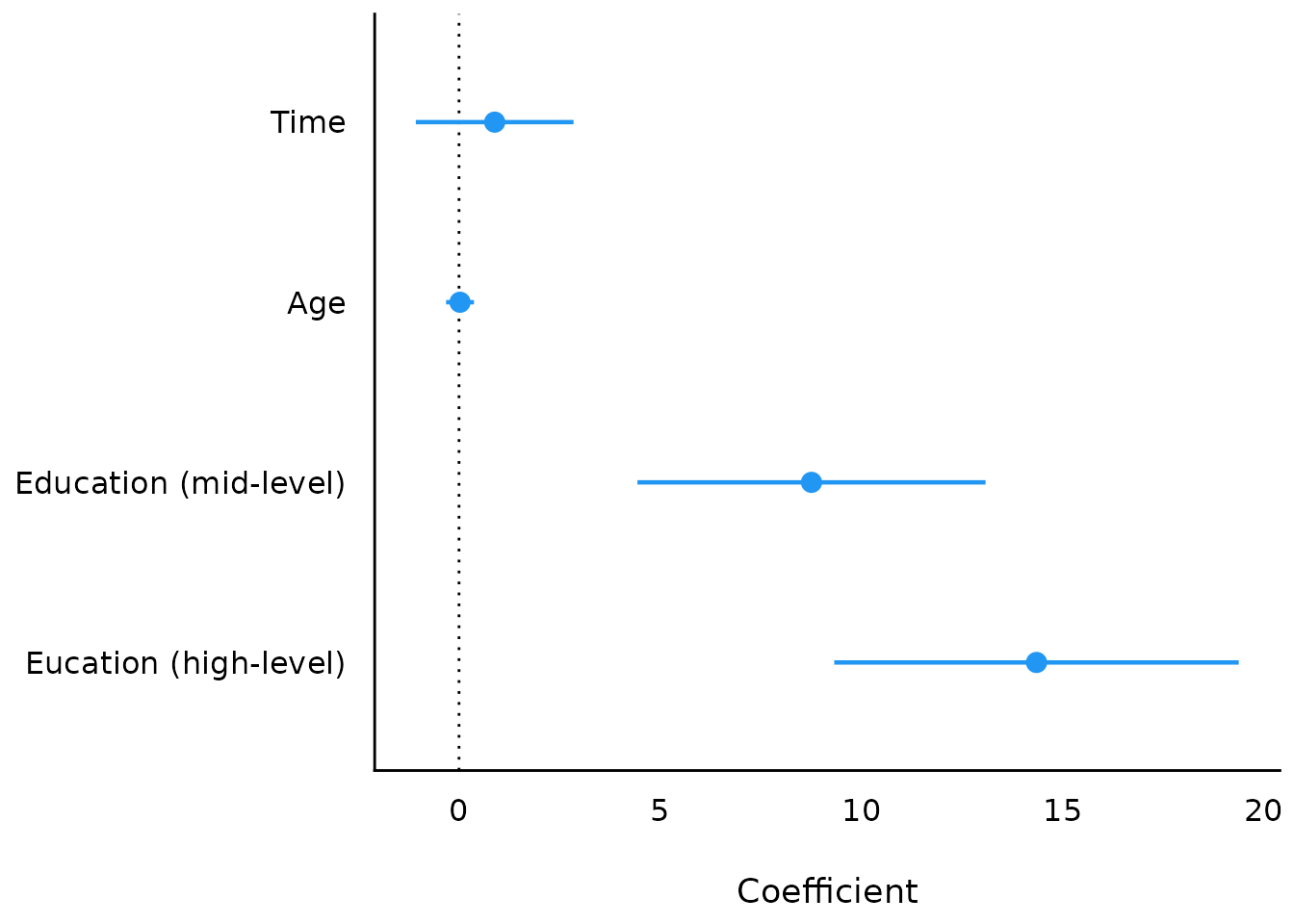## Simulated Model Parameters

simulate_parameters() computes simulated draws of parameters and their related indices such as Confidence Intervals (CI) and p-values. Simulating parameter draws can be seen as a (computationally faster) alternative to bootstrapping.

As simulate_parameters() is based on simulate_model() and thus simulates many draws for each parameter, plot() will produce similar plots as the density estimation plots from Bayesian models.

result <- simulate_parameters(model1)

plot(result)plot(result, stack = FALSE)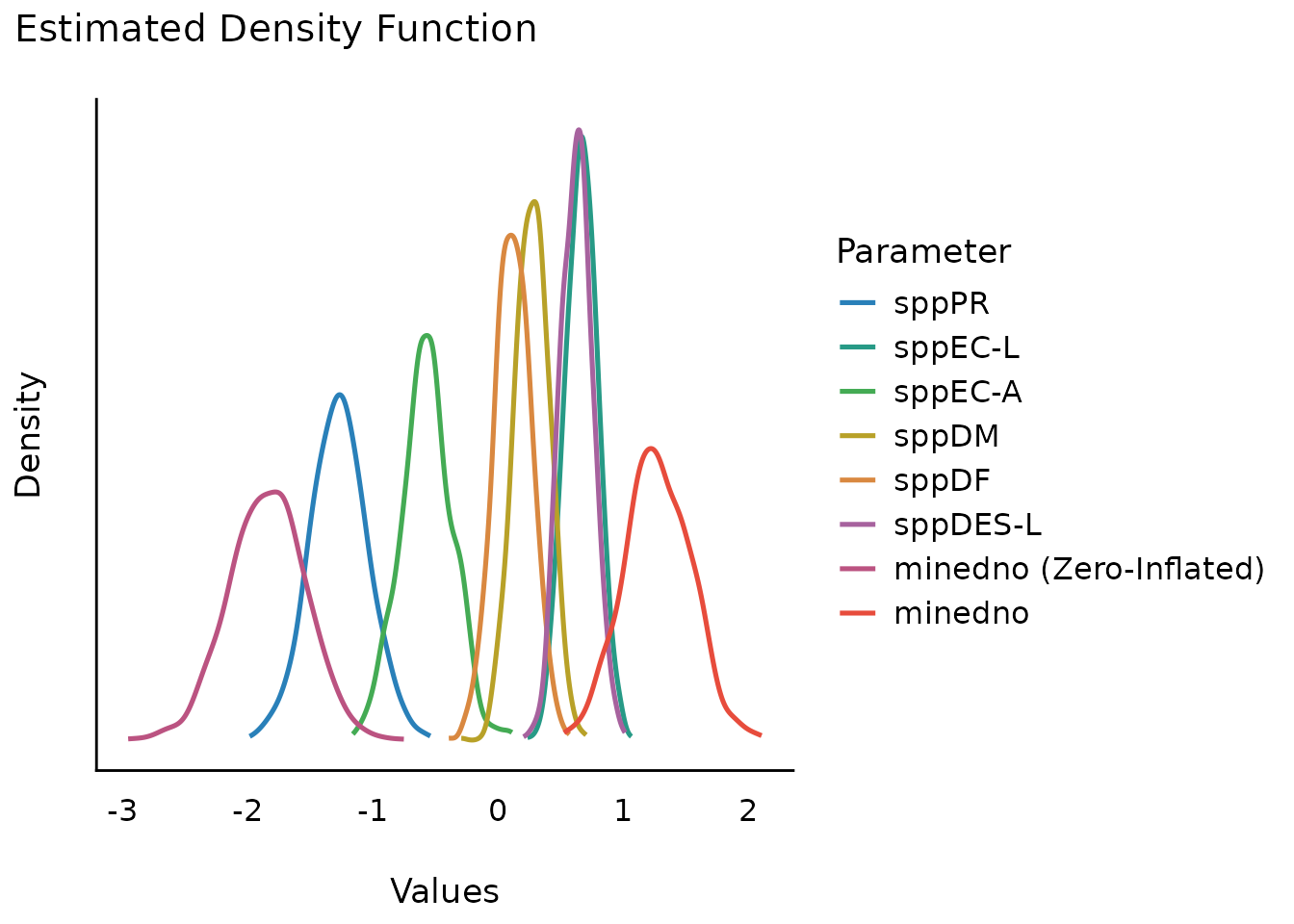To avoid vertical overlapping, use normalize_height.

plot(result, stack = FALSE, normalize_height = TRUE)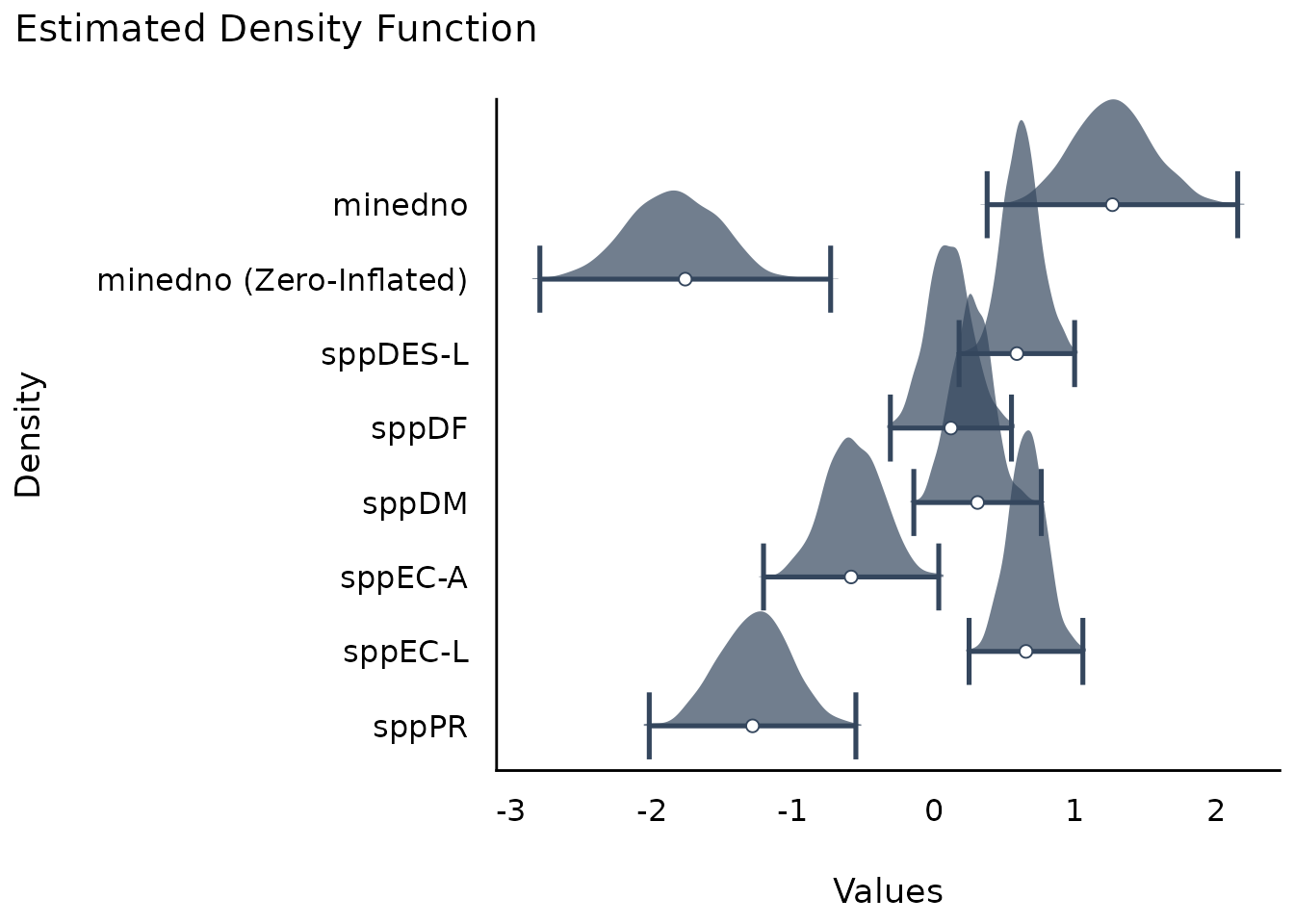plot(result, n_columns = 2)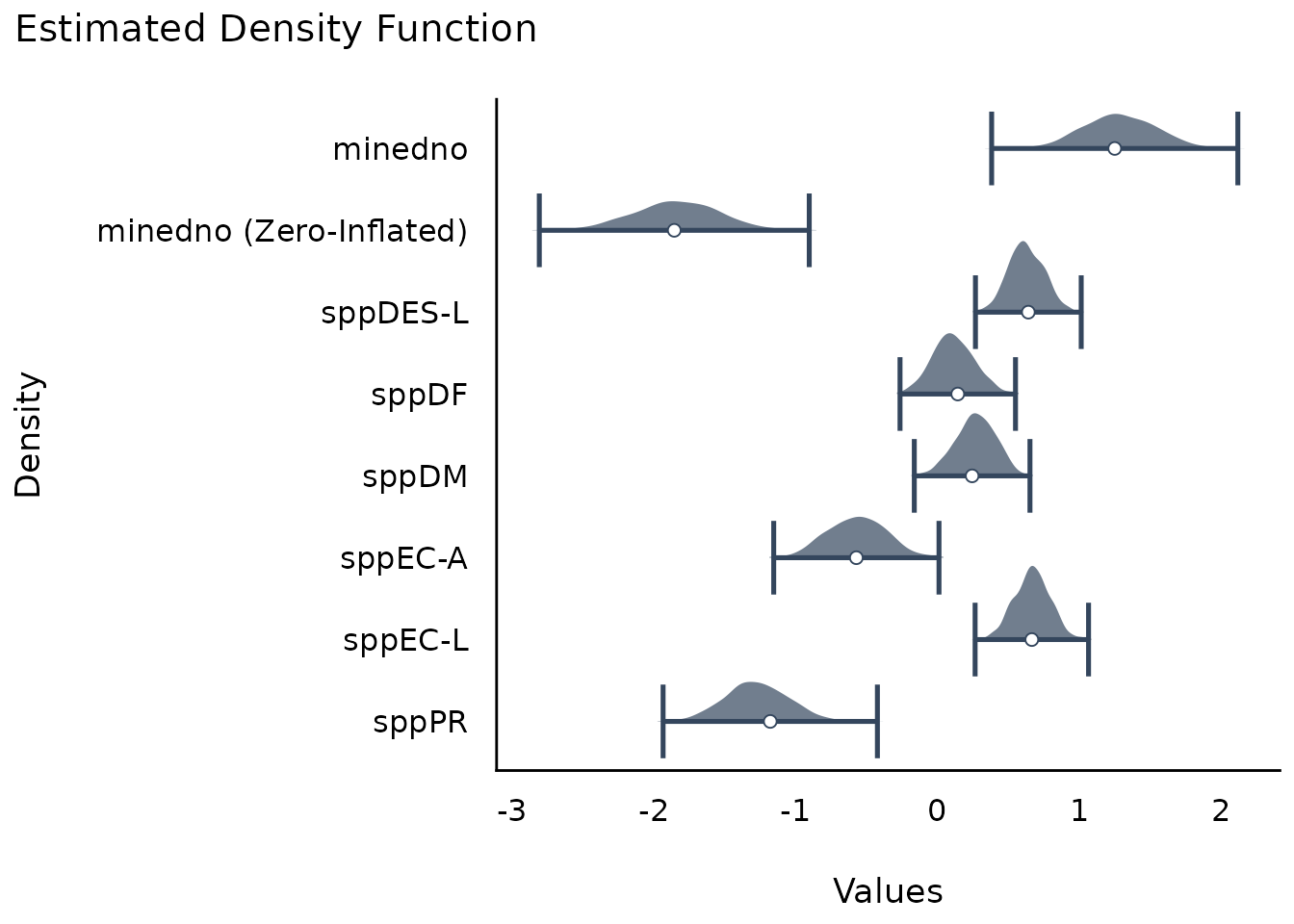plot(result, n_columns = 2, stack = FALSE)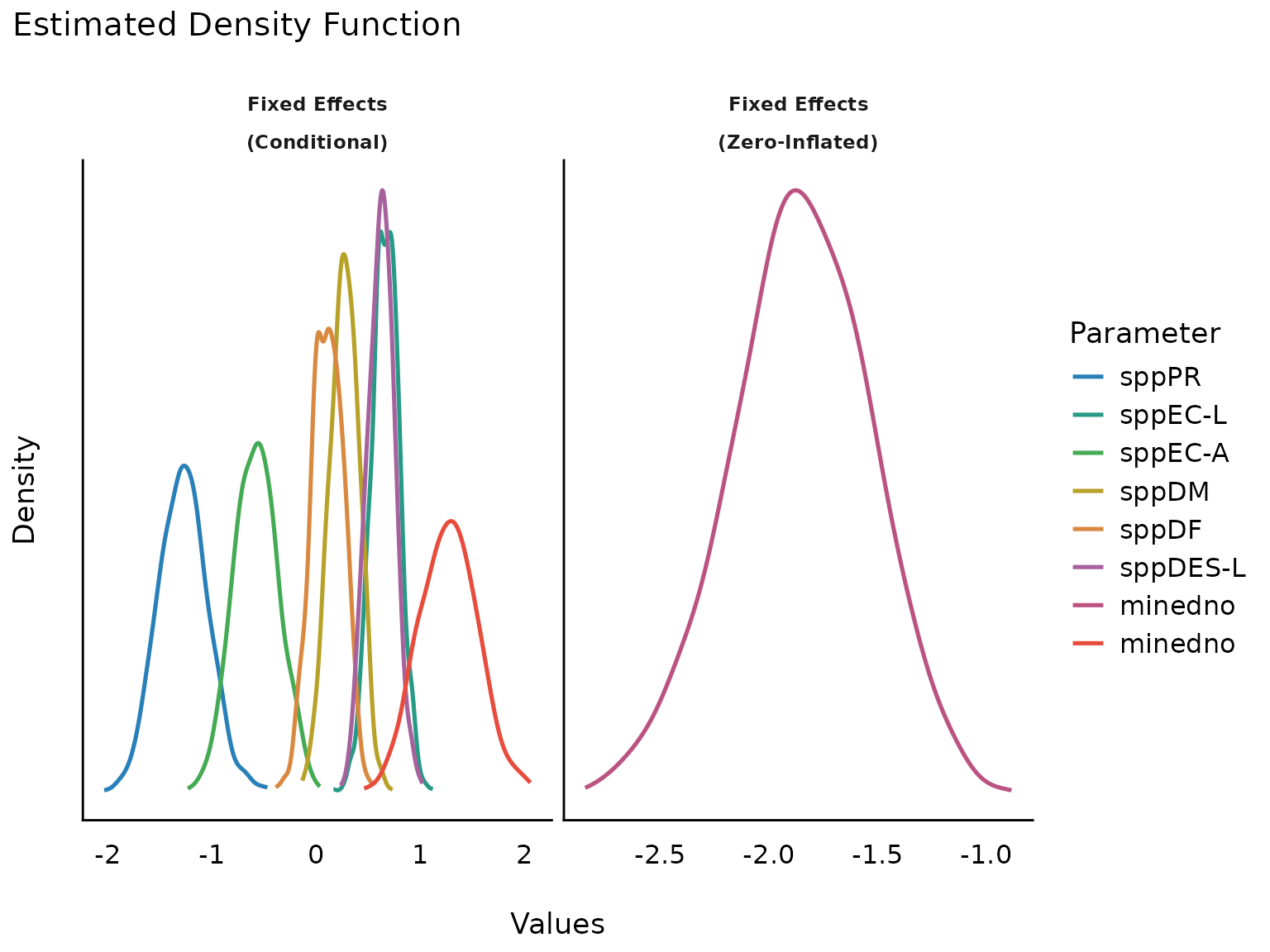## Model Parameters of SEM models

structure <- " visual  =~ x1 + x2 + x3
textual =~ x4 + x5 + x6
speed   =~ x7 + x8 + x9 "

model <- lavaan::cfa(structure, data = HolzingerSwineford1939)
result <- model_parameters(model)
plot(result)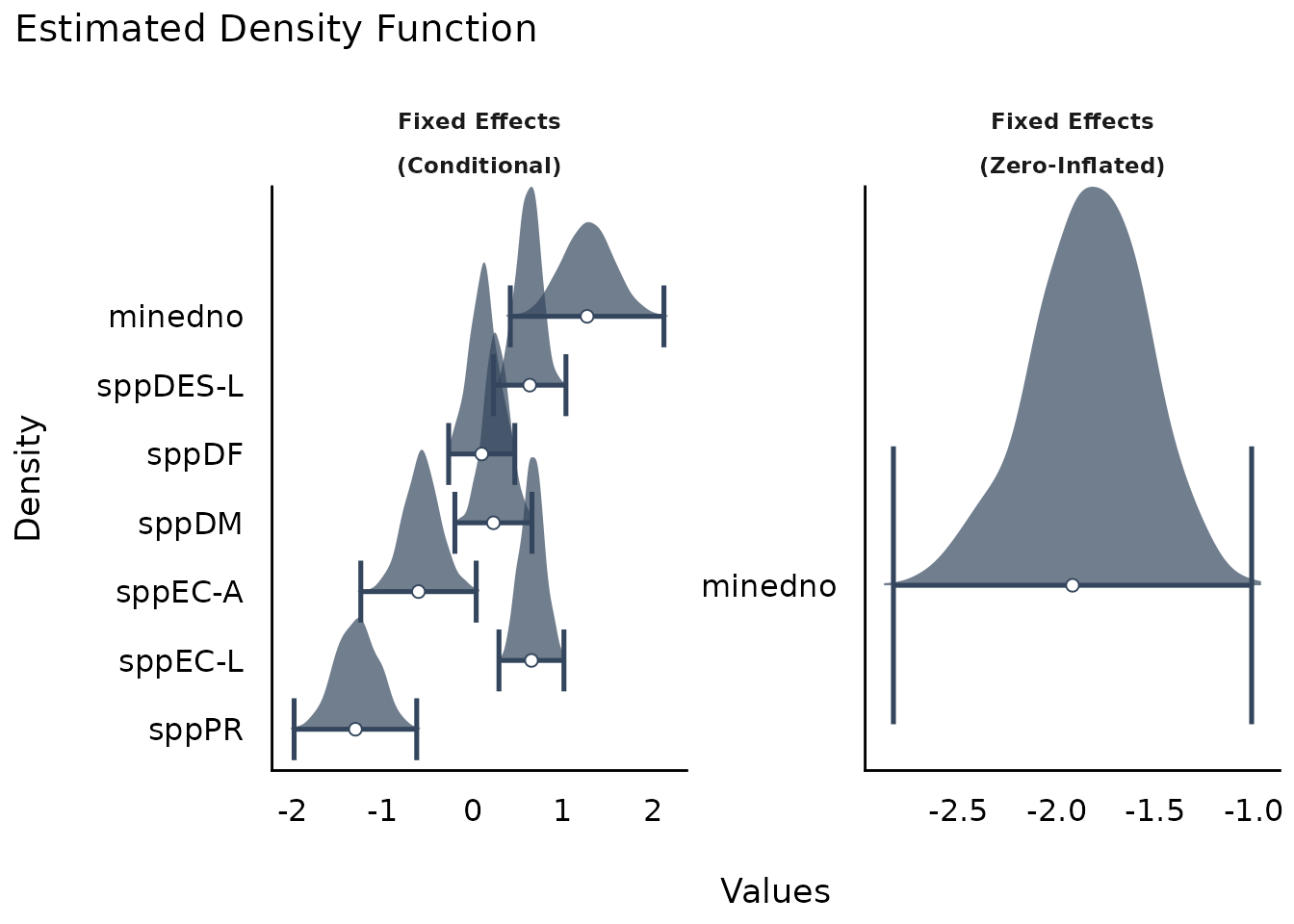## Model Parameters of Bayesian models

model_parameters() for Bayesian models will produce “forest plots” (instead of density estimations).

# We download the model to save computation time. Here is the code
# to refit the exact model used below...

# set.seed(123)
# model <- brm(bf(
#     count ~ persons + child + camper + (1 | persons),
#     zi ~ child + camper + (1 | persons)
#   ),
#   data = zinb,
#   family = zero_inflated_poisson()
# )
result <- model_parameters(brms_model, effects = "all", component = "all")

plot(result)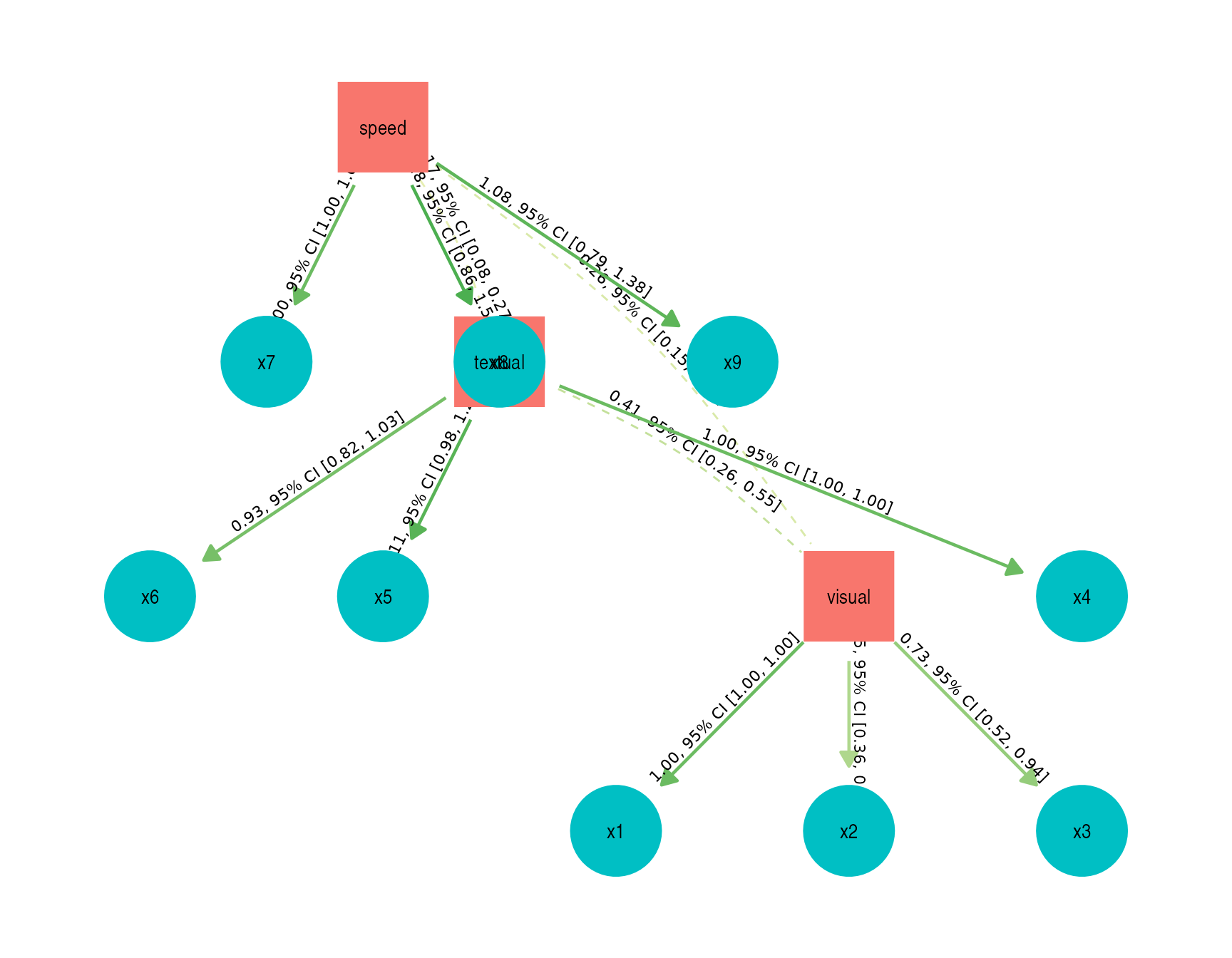### Including group levels of random effects

result <- model_parameters(brms_model,
effects = "all",
component = "all", group_level = TRUE
)
plot(result)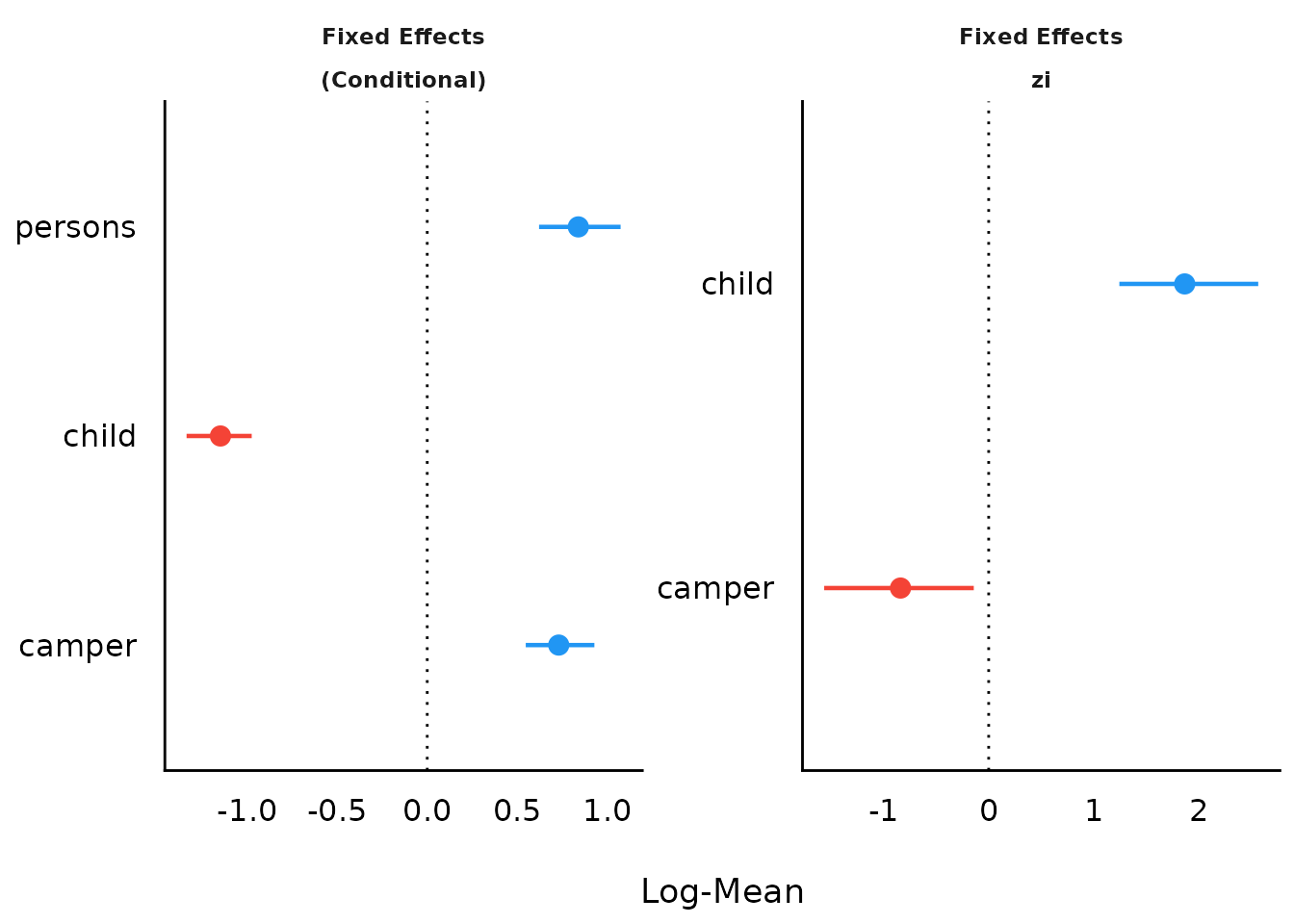### One-column Layout

plot(result, n_column = 1)### Including Intercepts and Variance Estimates for Random Intercepts

plot(result, show_intercept = TRUE)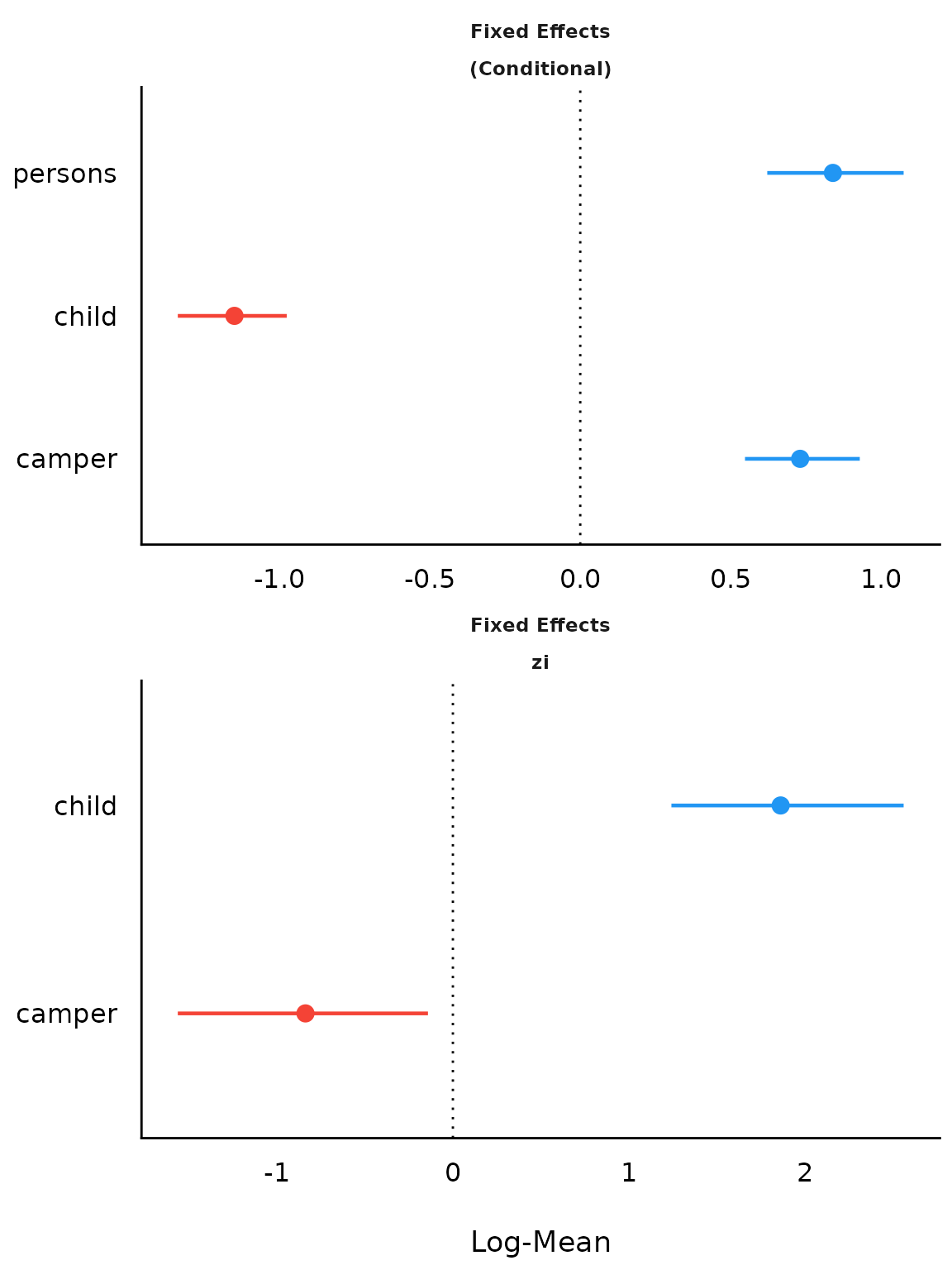## Model Parameters of Meta-Analysis models

mydat <- data.frame(
effectsize = c(-0.393, 0.675, 0.282, -1.398),
standarderror = c(0.317, 0.317, 0.13, 0.36)
)

ma <- rma(yi = effectsize, sei = standarderror, method = "REML", data = mydat)
result <- model_parameters(ma)

result
#> Meta-analysis using 'metafor'
#>
#> Parameter | Coefficient |   SE |         95% CI |     z |      p | Weight
#> -------------------------------------------------------------------------
#> Study 1   |       -0.39 | 0.32 | [-1.01,  0.23] | -1.24 | 0.215  |   9.95
#> Study 2   |        0.68 | 0.32 | [ 0.05,  1.30] |  2.13 | 0.033  |   9.95
#> Study 3   |        0.28 | 0.13 | [ 0.03,  0.54] |  2.17 | 0.030  |  59.17
#> Study 4   |       -1.40 | 0.36 | [-2.10, -0.69] | -3.88 | < .001 |   7.72
#> Overall   |       -0.18 | 0.44 | [-1.05,  0.68] | -0.42 | 0.676  |
plot(result)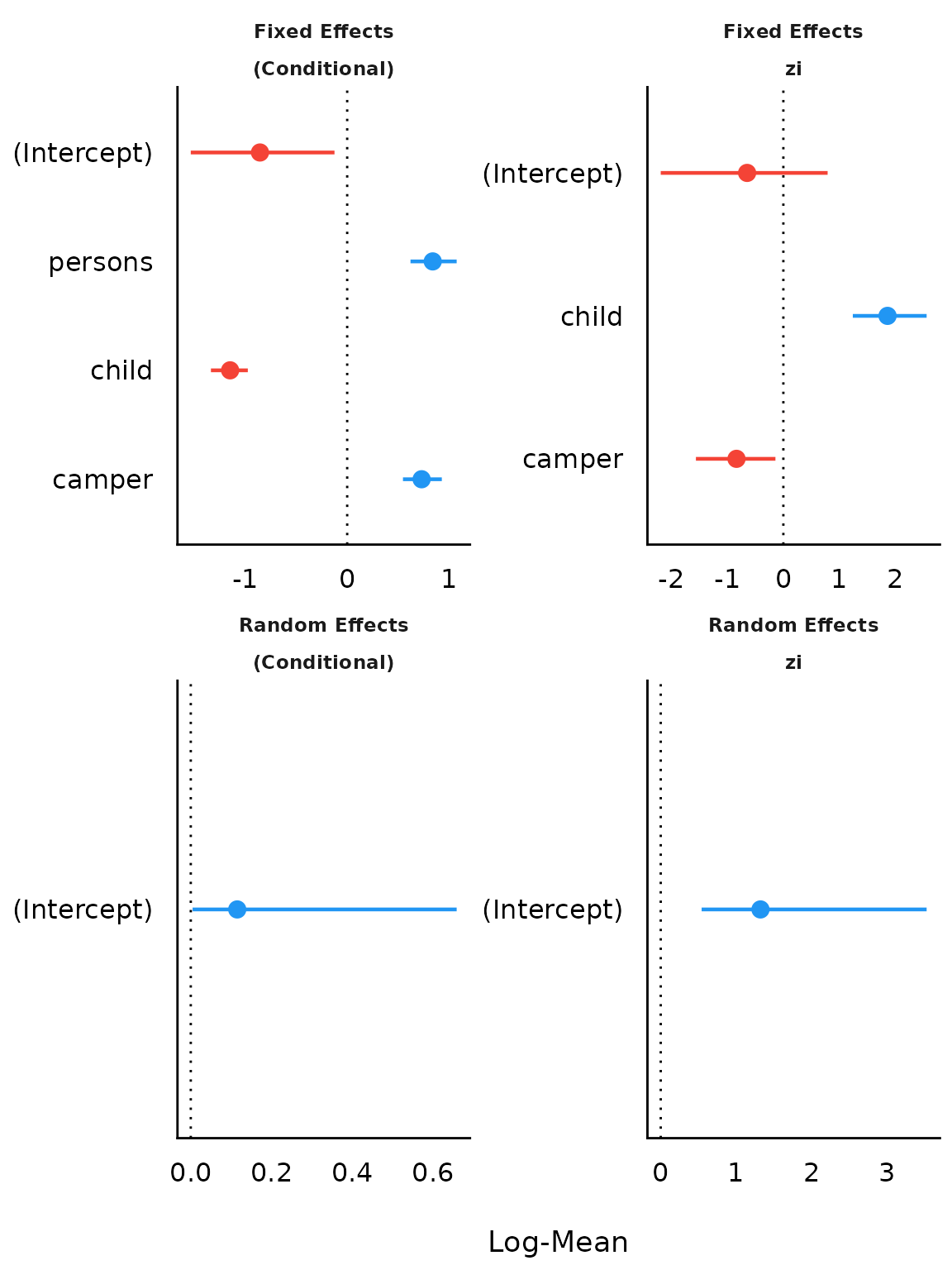If show_labels is TRUE, estimates and confidence intervals are included in the plot. Adjust the text size with size_text.

plot(result, show_labels = TRUE, size_text = 4)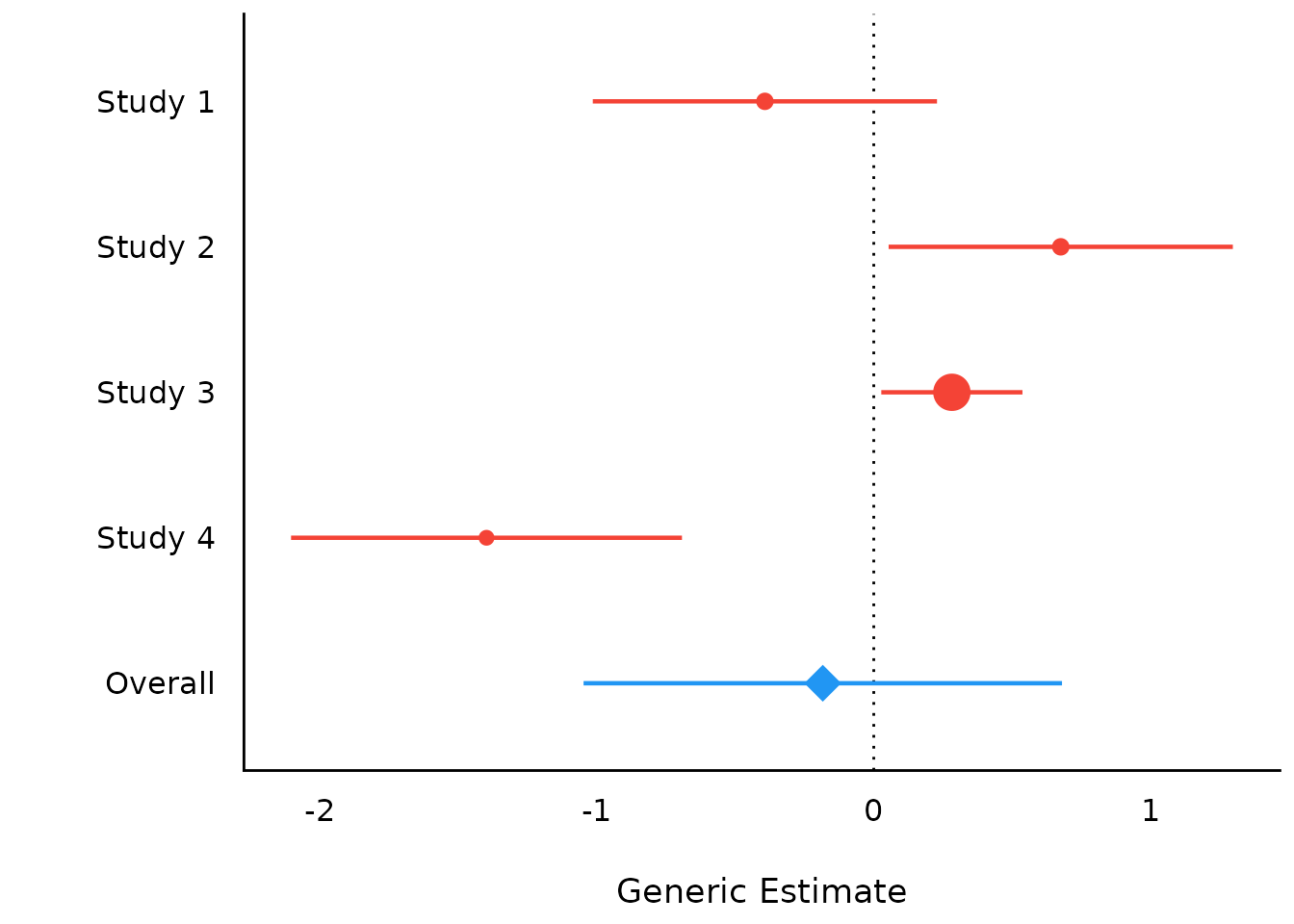### Funnel plots

If type = "funnel", a funnel plot is created.

plot(result, type = "funnel")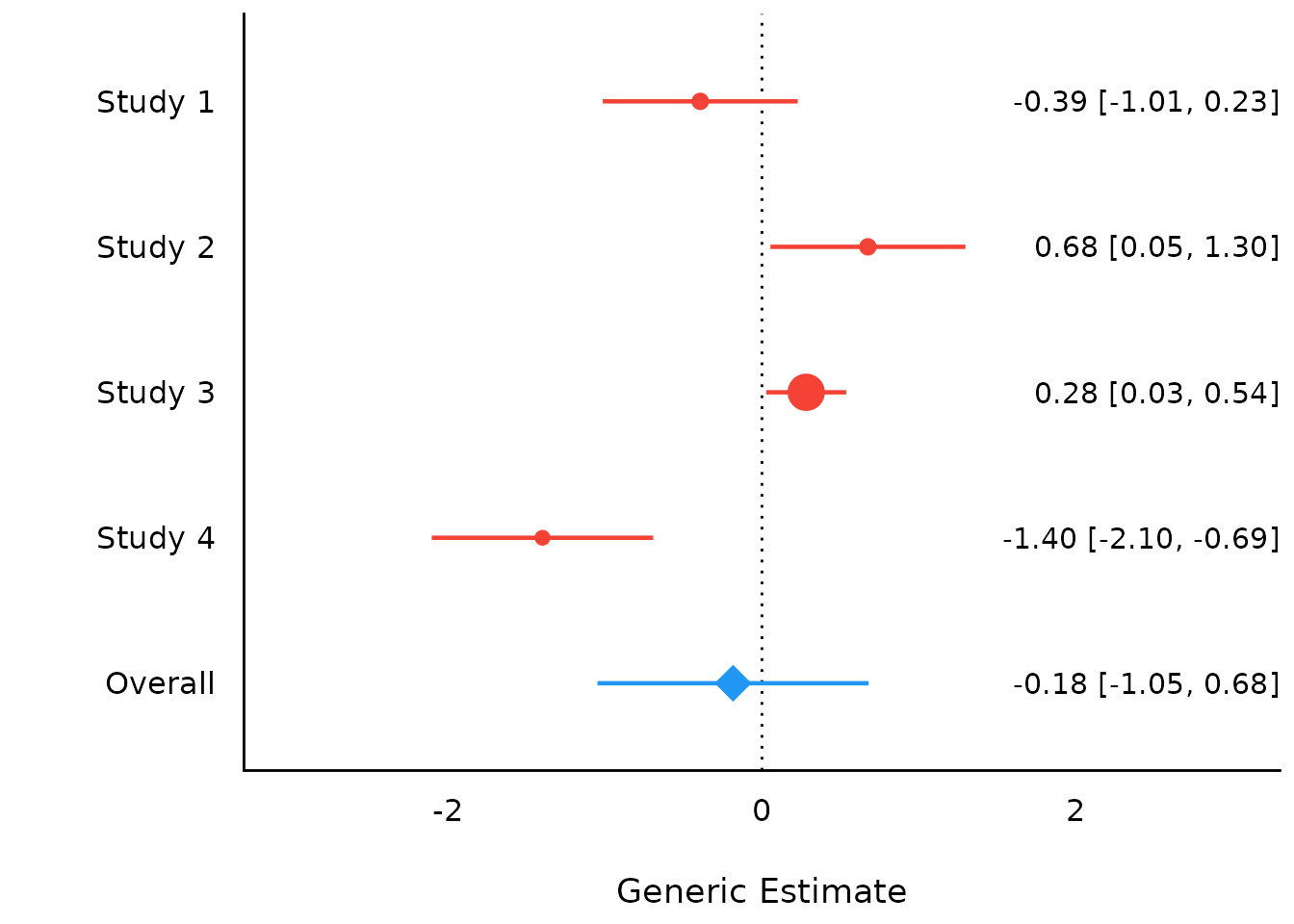### Model Parameters of Meta-Analysis Models with Subgroups

set.seed(123)
data(dat.bcg)
dat <- escalc(
measure = "RR",
ai = tpos,
bi = tneg,
ci = cpos,
di = cneg,
data = dat.bcg
)
dat$author <- make.unique(dat$author)
dat$disease <- sample(c("Cancer", "CVD", "Depression"), size = nrow(dat), replace = TRUE) model <- rma(yi, vi, mods = ~disease, data = dat, digits = 3, slab = author) result <- model_parameters(model) result #> # Depression #> #> Parameter | Coefficient | SE | 95% CI | z | p | Weight #> ------------------------------------------------------------------------------------ #> Aronson | -0.89 | 0.57 | [-2.01, 0.23] | -1.56 | 0.119 | 3.07 #> Ferguson & Simes | -1.59 | 0.44 | [-2.45, -0.72] | -3.59 | < .001 | 5.14 #> Rosenthal et al | -1.35 | 0.64 | [-2.61, -0.08] | -2.09 | 0.036 | 2.41 #> Frimodt-Moller et al | -0.22 | 0.23 | [-0.66, 0.23] | -0.96 | 0.336 | 19.53 #> Coetzee & Berjak | -0.47 | 0.24 | [-0.94, 0.00] | -1.98 | 0.048 | 17.72 #> Overall | -0.12 | 0.59 | [-1.28, 1.04] | -0.20 | 0.841 | #> #> # CVD #> #> Parameter | Coefficient | SE | 95% CI | z | p | Weight #> ----------------------------------------------------------------------------------- #> Hart & Sutherland | -1.44 | 0.14 | [-1.72, -1.16] | -10.19 | < .001 | 49.97 #> Stein & Aronson | -0.79 | 0.08 | [-0.95, -0.62] | -9.46 | < .001 | 144.81 #> Vandiviere et al | -1.62 | 0.47 | [-2.55, -0.70] | -3.43 | < .001 | 4.48 #> TPT Madras | 0.01 | 0.06 | [-0.11, 0.14] | 0.19 | 0.849 | 252.42 #> Comstock et al | -0.34 | 0.11 | [-0.56, -0.12] | -3.05 | 0.002 | 80.57 #> Comstock & Webster | 0.45 | 0.73 | [-0.98, 1.88] | 0.61 | 0.541 | 1.88 #> Overall | 0.03 | 0.56 | [-1.08, 1.13] | 0.05 | 0.963 | #> #> # Cancer #> #> Parameter | Coefficient | SE | 95% CI | z | p | Weight #> --------------------------------------------------------------------------------- #> Rosenthal et al.1 | -1.37 | 0.27 | [-1.90, -0.84] | -5.07 | < .001 | 13.69 #> Comstock et al.1 | -0.02 | 0.27 | [-0.54, 0.51] | -0.06 | 0.948 | 14.00 #> Overall | -0.69 | 0.49 | [-1.65, 0.26] | -1.42 | 0.155 | plot(result)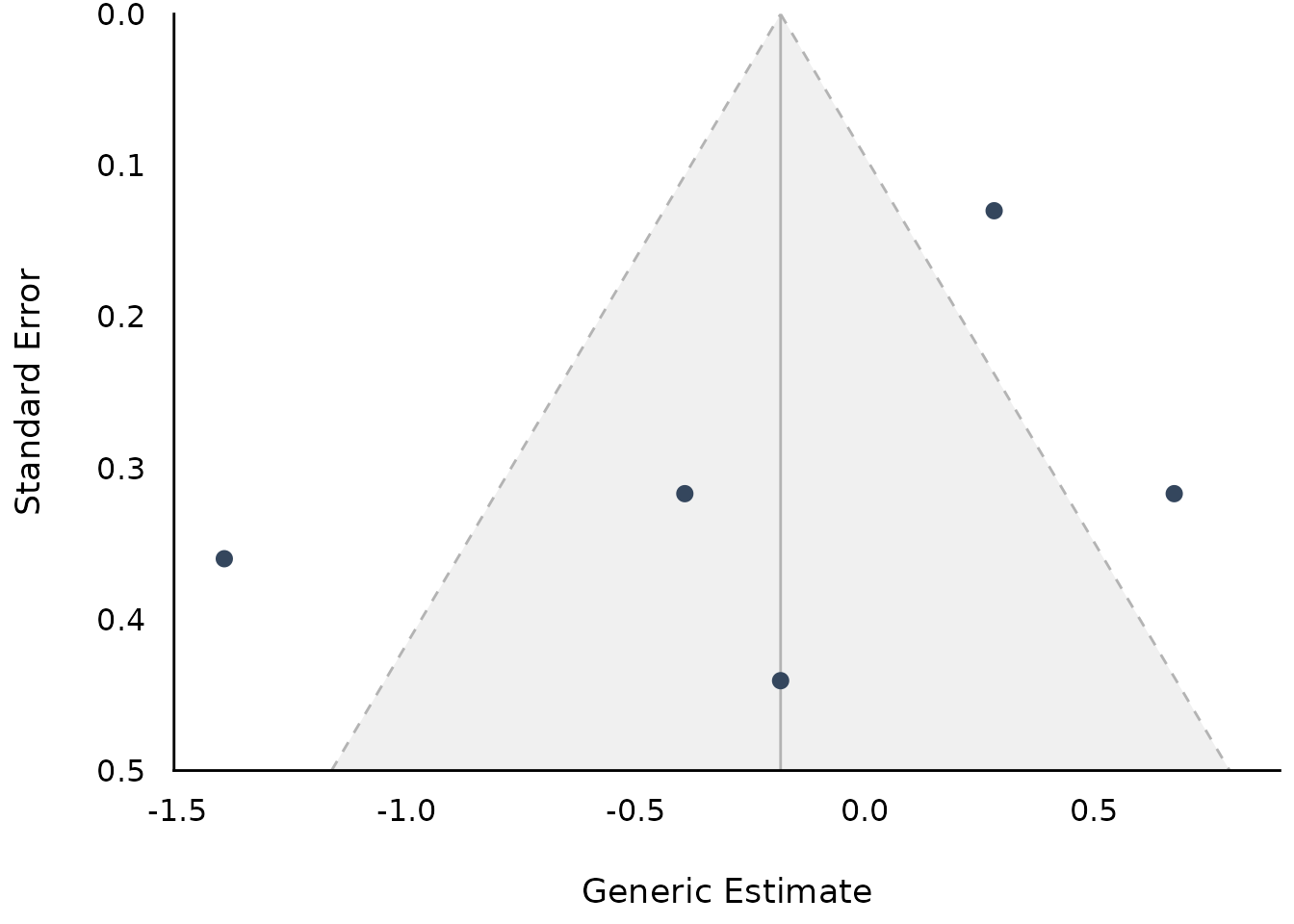## Bayesian Meta-Analysis using brms # We download the model to save computation time. Here is the code # to refit the exact model used below... # Data from # https://github.com/MathiasHarrer/Doing-Meta-Analysis-in-R/blob/master/_data/Meta_Analysis_Data.RData # set.seed(123) # priors <- c(prior(normal(0,1), class = Intercept), # prior(cauchy(0,0.5), class = sd)) # # model <- # brm(TE|se(seTE) ~ 1 + (1|Author), # data = Meta_Analysis_Data, # prior = priors, # iter = 4000) library(brms) model <- insight::download_model("brms_meta_1") result <- model_parameters(model) result plot(result) ## Comparison of Models (related function documentation) data(iris) # shorter variable name iris$Length <- iris$Petal.Length lm1 <- lm(Sepal.Length ~ Species, data = iris) lm2 <- lm(Sepal.Length ~ Species + Length, data = iris) lm3 <- lm(Sepal.Length ~ Species * Length, data = iris) result <- compare_parameters(lm1, lm2, lm3) plot(result)plot(result, size_text = 3.8) + labs(y = NULL) + theme(legend.position = "bottom")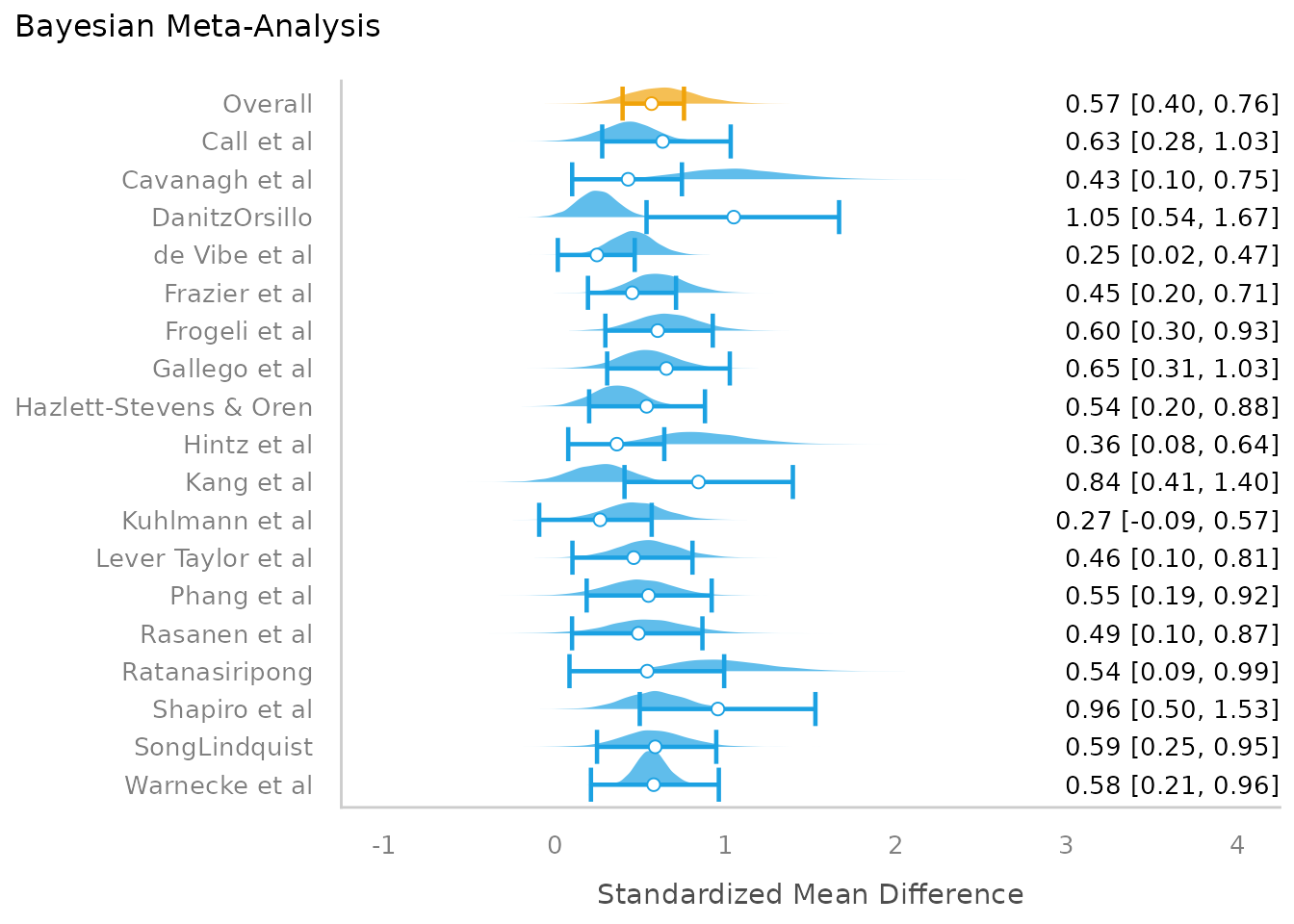## Equivalence Testing (related function documentation) ### For fixed effects # default rules, like in bayestestR::equivalence_test() result <- equivalence_test(model4) result #> # TOST-test for Practical Equivalence #> #> ROPE: [-1.99 1.99] #> #> Parameter | 90% CI | % in ROPE | H0 | p #> ------------------------------------------------------------------ #> (Intercept) | [59.33, 68.41] | 0% | Rejected | > .999 #> time | [-0.76, 2.53] | 83.52% | Undecided | 0.137 #> age | [-0.26, 0.32] | 100% | Accepted | < .001 #> education [mid] | [ 5.13, 12.39] | 0% | Rejected | 0.999 #> education [high] | [10.14, 18.57] | 0% | Rejected | > .999 plot(result)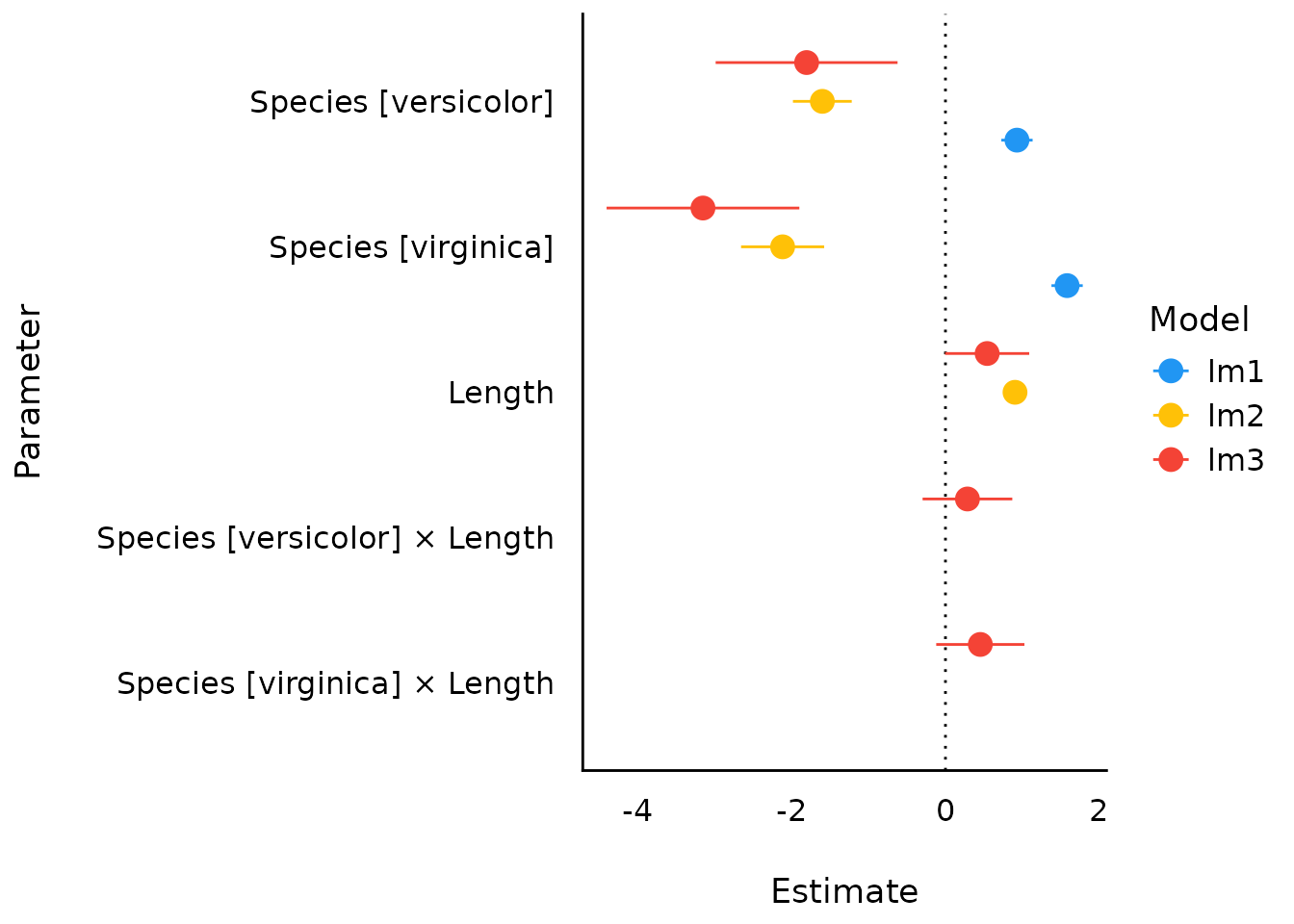result <- equivalence_test(model4, rule = "cet") result #> # Conditional Equivalence Testing #> #> ROPE: [-1.99 1.99] #> #> Parameter | 90% CI | % in ROPE | H0 | p #> ------------------------------------------------------------------ #> (Intercept) | [59.33, 68.41] | 0% | Rejected | > .999 #> time | [-0.76, 2.53] | 83.52% | Undecided | 0.137 #> age | [-0.26, 0.32] | 100% | Accepted | < .001 #> education [mid] | [ 5.13, 12.39] | 0% | Rejected | 0.999 #> education [high] | [10.14, 18.57] | 0% | Rejected | > .999 plot(result)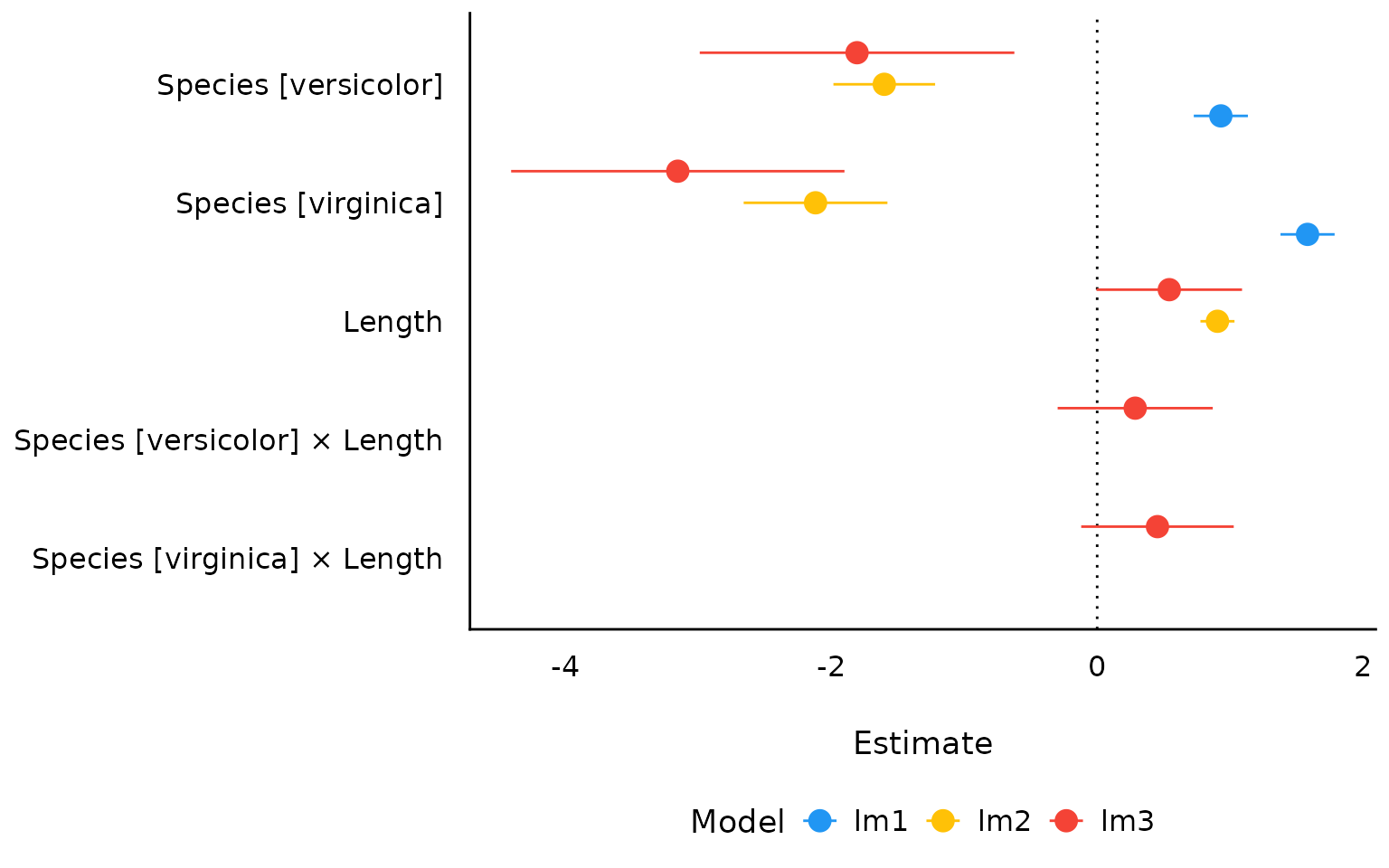### For random effects result <- equivalence_test(model3, effects = "random") result #> # TOST-test for Practical Equivalence #> #> ROPE: [-5.63 5.63] #> #> Group: grp #> #> Parameter | Estimate | Group | 90% CI | % in ROPE | H0 #> ---------------------------------------------------------------------- #> 1 | 1.18 | grp | [ -1.91, 4.28] | 100% | Accepted #> 2 | -0.21 | grp | [ -3.27, 2.84] | 100% | Accepted #> 3 | -0.25 | grp | [ -3.33, 2.83] | 100% | Accepted #> 4 | -0.48 | grp | [ -3.56, 2.60] | 100% | Accepted #> 5 | -0.24 | grp | [ -3.33, 2.85] | 100% | Accepted #> Group: Subject #> #> Parameter | Estimate | Group | 90% CI | % in ROPE | H0 #> ------------------------------------------------------------------------- #> 308 | 40.86 | Subject | [ 25.22, 56.50] | 0% | Rejected #> 309 | -77.88 | Subject | [-93.51, -62.24] | 0% | Rejected #> 310 | -63.28 | Subject | [-78.91, -47.65] | 0% | Rejected #> 330 | 4.32 | Subject | [-11.32, 19.96] | 36.01% | Undecided #> 331 | 10.18 | Subject | [ -5.45, 25.80] | 35.46% | Undecided #> 332 | 8.47 | Subject | [ -7.17, 24.10] | 36.02% | Undecided #> 333 | 16.44 | Subject | [ 0.81, 32.07] | 15.44% | Rejected #> 334 | -3.00 | Subject | [-18.65, 12.65] | 36.00% | Undecided #> 335 | -45.48 | Subject | [-61.11, -29.85] | 0% | Rejected #> 337 | 72.16 | Subject | [ 56.54, 87.79] | 0% | Rejected #> 349 | -20.91 | Subject | [-36.57, -5.25] | 1.22% | Rejected #> 350 | 14.23 | Subject | [ -1.41, 29.88] | 22.52% | Undecided #> 351 | -8.07 | Subject | [-23.74, 7.59] | 35.97% | Undecided #> 352 | 36.44 | Subject | [ 20.76, 52.12] | 0% | Rejected #> 369 | 6.97 | Subject | [ -8.66, 22.60] | 36.05% | Undecided #> 370 | -6.31 | Subject | [-21.96, 9.34] | 35.99% | Undecided #> 371 | -3.34 | Subject | [-18.96, 12.29] | 36.05% | Undecided #> 372 | 18.21 | Subject | [ 2.57, 33.84] | 9.80% | Rejected plot(result)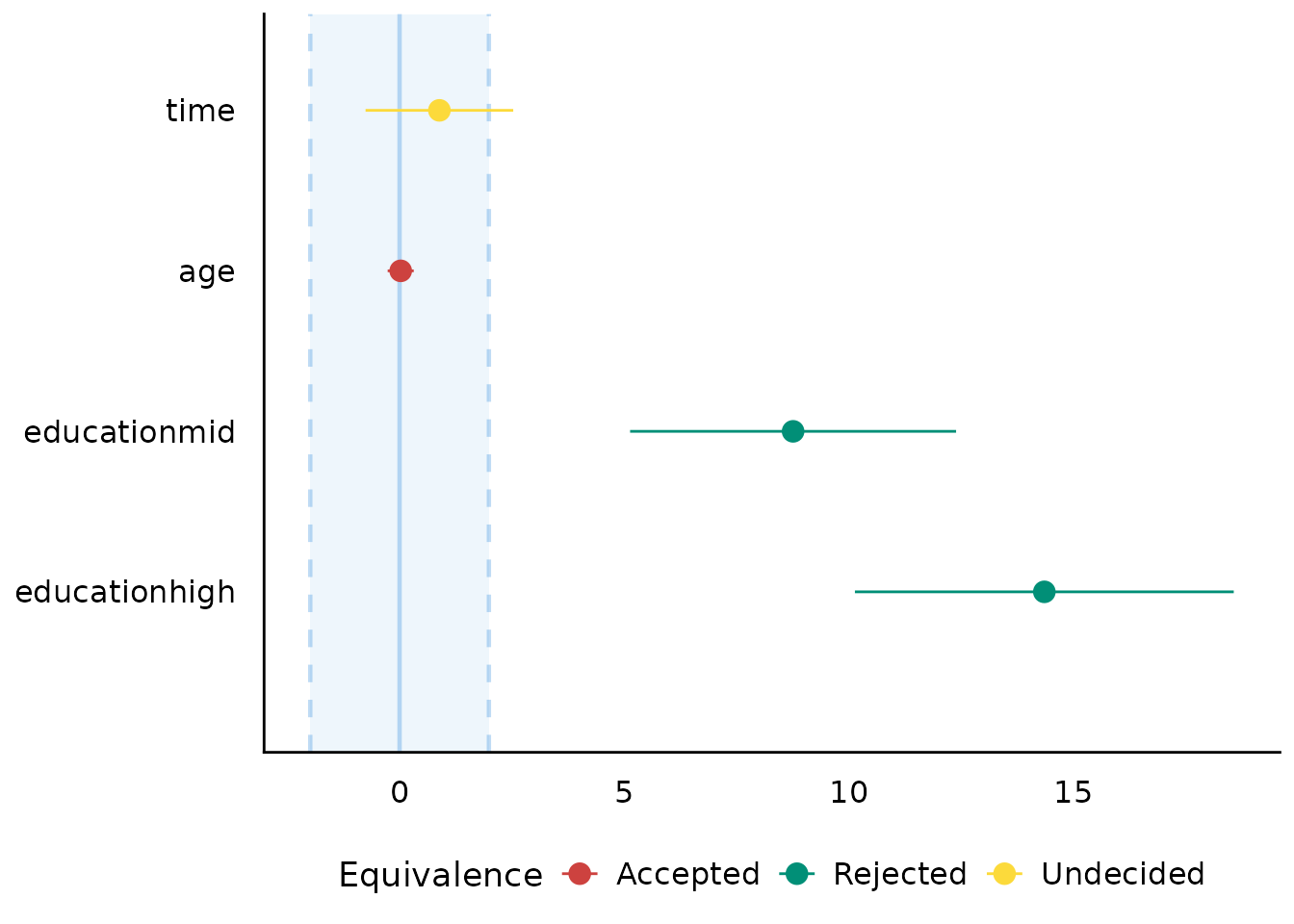### From simulated model parameters simulated_parameters <- simulate_model(model1, component = "conditional") result <- equivalence_test(simulated_parameters) result #> # Test for Practical Equivalence #> #> ROPE: [-0.10 0.10] #> #> Parameter | H0 | inside ROPE | 95% HDI #> ----------------------------------------------------- #> (Intercept) | Undecided | 12.42 % | [-0.91 0.19] #> sppPR | Rejected | 0.00 % | [-1.72 -0.81] #> sppDM | Undecided | 9.79 % | [ 0.00 0.54] #> sppEC-A | Rejected | 0.00 % | [-0.95 -0.17] #> sppEC-L | Rejected | 0.00 % | [ 0.41 0.91] #> sppDES-L | Rejected | 0.00 % | [ 0.39 0.86] #> sppDF | Undecided | 38.74 % | [-0.15 0.38] #> minedno | Rejected | 0.00 % | [ 0.70 1.82] plot(result)## Principal Component Analysis (related function documentation) data(mtcars) result <- principal_components(mtcars[, 1:7], n = "all", threshold = 0.2) result #> # Loadings from Principal Component Analysis (no rotation) #> #> Variable | PC1 | PC2 | PC3 | PC4 | PC5 | PC6 | Complexity #> ------------------------------------------------------------------- #> mpg | -0.93 | | | -0.30 | | | 1.30 #> cyl | 0.96 | | | | | -0.21 | 1.18 #> disp | 0.95 | | | -0.23 | | | 1.16 #> hp | 0.87 | 0.36 | | | 0.30 | | 1.64 #> drat | -0.75 | 0.48 | 0.44 | | | | 2.47 #> wt | 0.88 | -0.35 | 0.26 | | | | 1.54 #> qsec | -0.54 | -0.81 | | | | | 1.96 #> #> The 6 principal components accounted for 99.30% of the total variance of the original data (PC1 = 72.66%, PC2 = 16.52%, PC3 = 4.93%, PC4 = 2.26%, PC5 = 1.85%, PC6 = 1.08%). plot(result)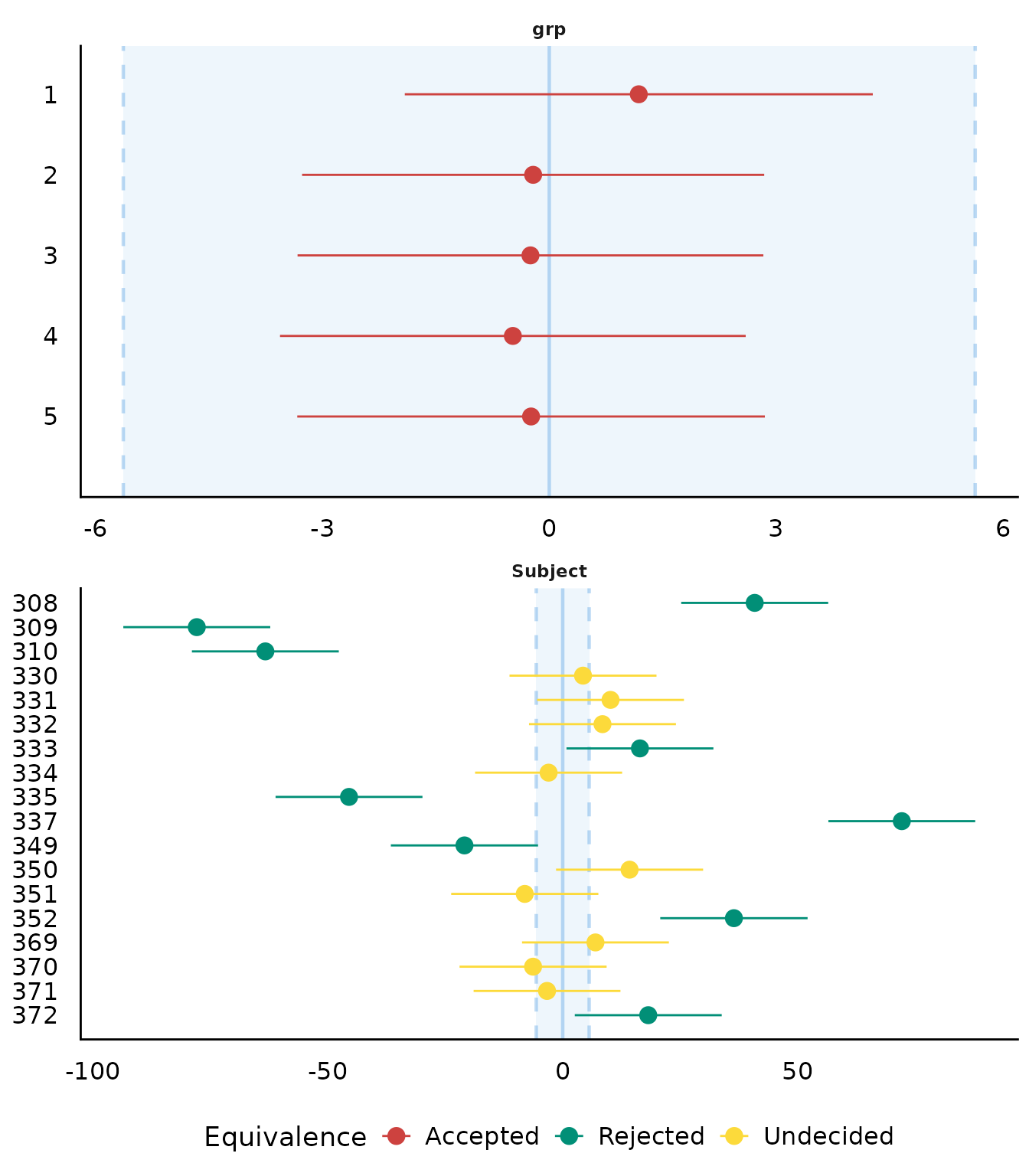result <- principal_components( mtcars[, 1:7], n = 3, rotation = "varimax", threshold = "max", sort = TRUE ) result #> # Rotated loadings from Principal Component Analysis (varimax-rotation) #> #> Variable | RC1 | RC2 | RC3 | Complexity | Uniqueness | MSA #> ----------------------------------------------------------------- #> wt | 0.91 | | | 1.31 | 0.03 | 0.77 #> mpg | -0.82 | | | 1.70 | 0.11 | 0.87 #> disp | 0.79 | | | 1.95 | 0.08 | 0.85 #> cyl | 0.64 | | | 2.84 | 0.06 | 0.87 #> qsec | | -0.98 | | 1.02 | 0.03 | 0.61 #> hp | | 0.69 | | 2.09 | 0.09 | 0.90 #> drat | | | -0.90 | 1.43 | 0.01 | 0.85 #> #> The 3 principal components (varimax rotation) accounted for 94.11% of the total variance of the original data (RC1 = 45.02%, RC2 = 27.79%, RC3 = 21.30%). plot(result, type = "line", text_color = "white") + theme_abyss()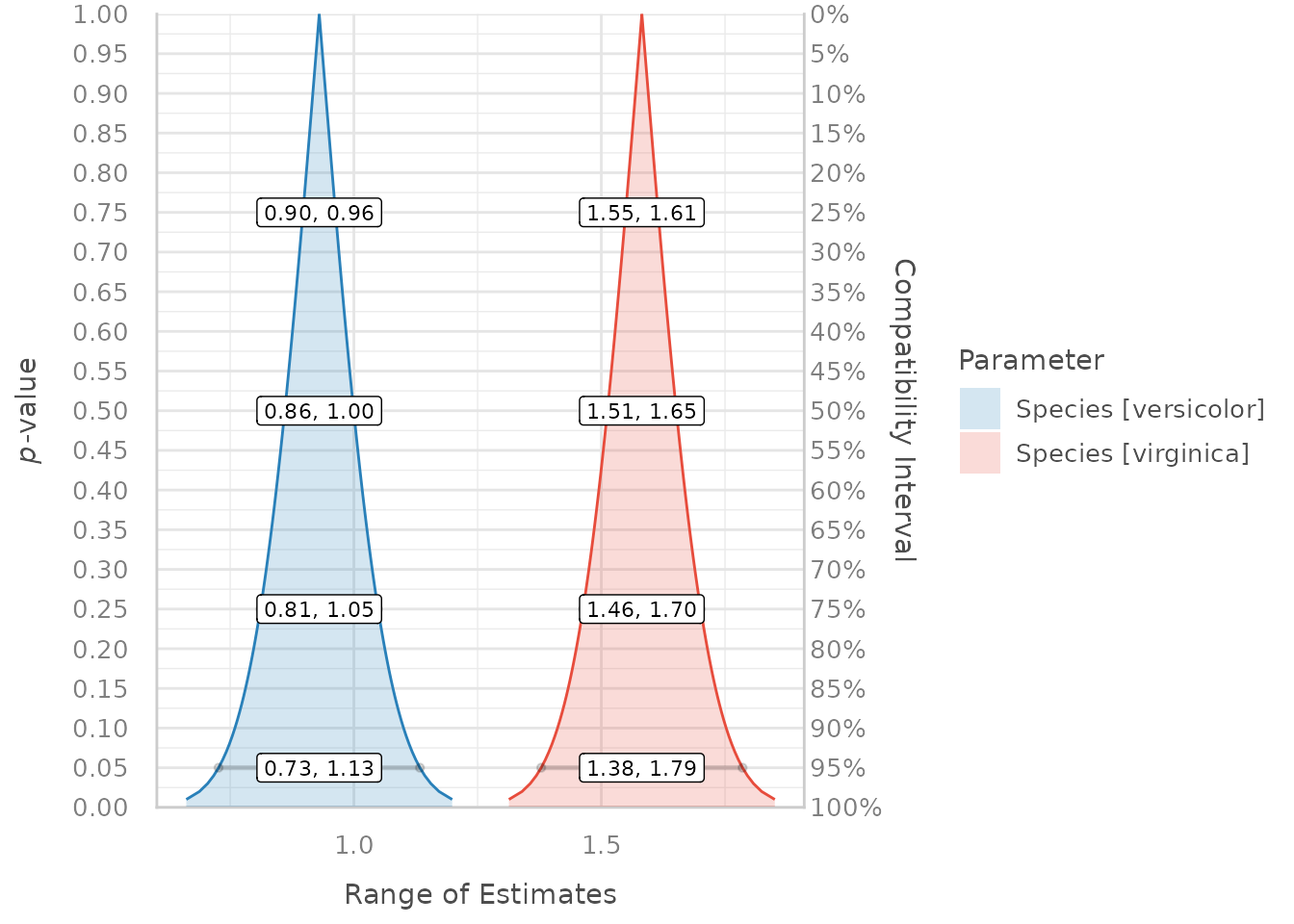## Cluster Analysis (related function documentation) ### Clustering solution data(iris) result <- cluster_analysis(iris[, 1:4], n = 3) result #> # Clustering Solution #> #> The 3 clusters accounted for 76.70% of the total variance of the original data. #> #> Cluster | n_Obs | Sum_Squares | Sepal.Length | Sepal.Width | Petal.Length | Petal.Width #> --------------------------------------------------------------------------------------- #> 1 | 47 | 47.45 | 1.13 | 0.09 | 0.99 | 1.01 #> 2 | 50 | 47.35 | -1.01 | 0.85 | -1.30 | -1.25 #> 3 | 53 | 44.09 | -0.05 | -0.88 | 0.35 | 0.28 #> #> Sum_Squares_Total Sum_Squares_Between Sum_Squares_Within R2 #> 1 596 457.1116 138.8884 0.7669658 #> #> # You can access the predicted clusters via 'predict()'. plot(result)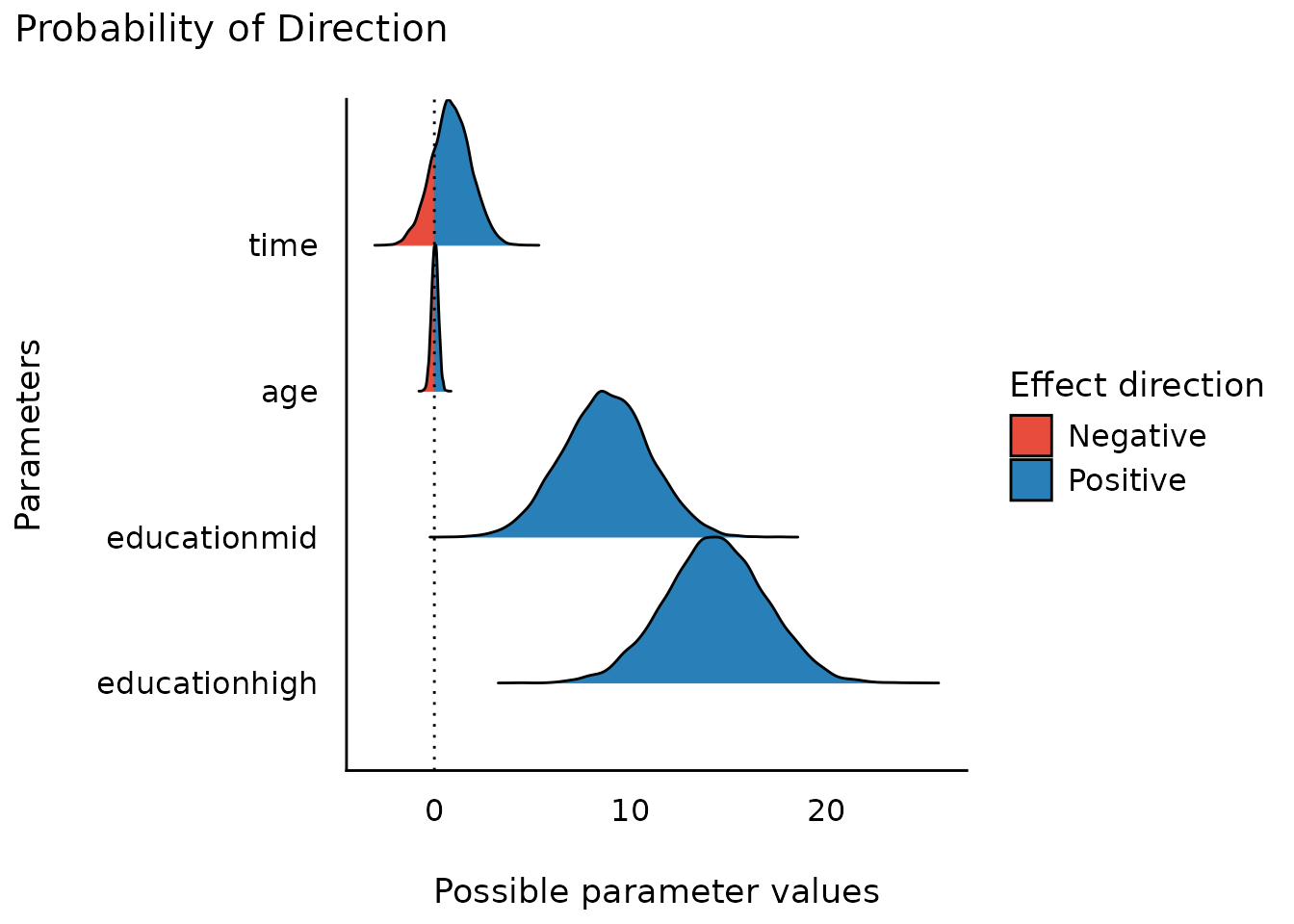result <- cluster_analysis(iris[, 1:4], n = 4) plot(result)### Cluster centers s <- summary(result) s #> Cluster Sepal.Length Sepal.Width Petal.Length Petal.Width #> 1 1 -0.05005221 -0.88042696 0.3465767 0.2805873 #> 2 2 1.13217737 0.08812645 0.9928284 1.0141287 #> 3 3 -0.62595645 1.80426129 -1.2826445 -1.2290567 #> 4 4 -1.19247841 0.40154427 -1.3090939 -1.2608902 plot(s)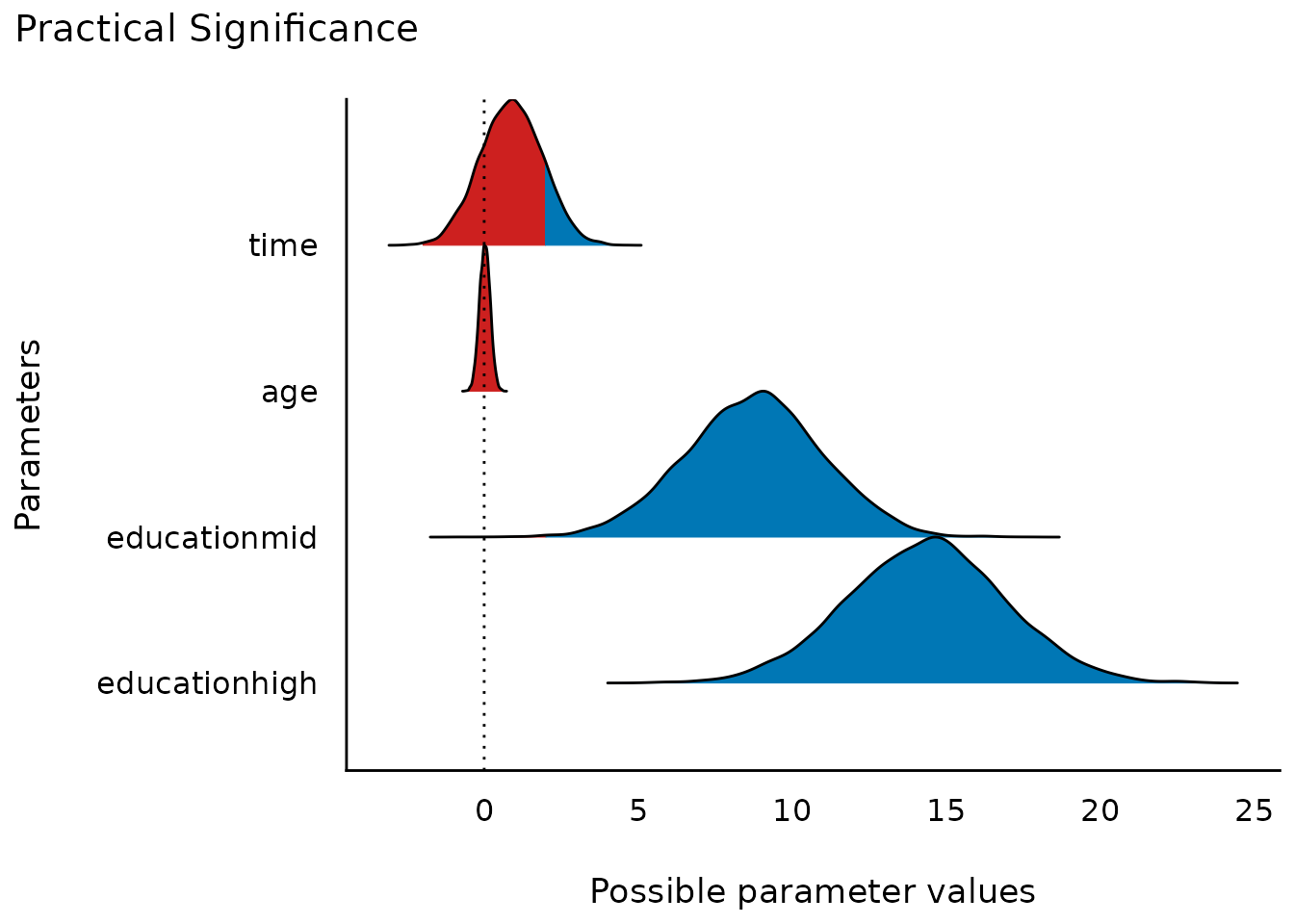## Number of Components/Factors to Retain (related function documentation) data(mtcars) result <- n_factors(mtcars, type = "PCA") result #> # Method Agreement Procedure: #> #> The choice of 3 dimensions is supported by 5 (29.41%) methods out of 17 (Bartlett, CNG, Scree (SE), Scree (R2), Velicer's MAP). plot(result)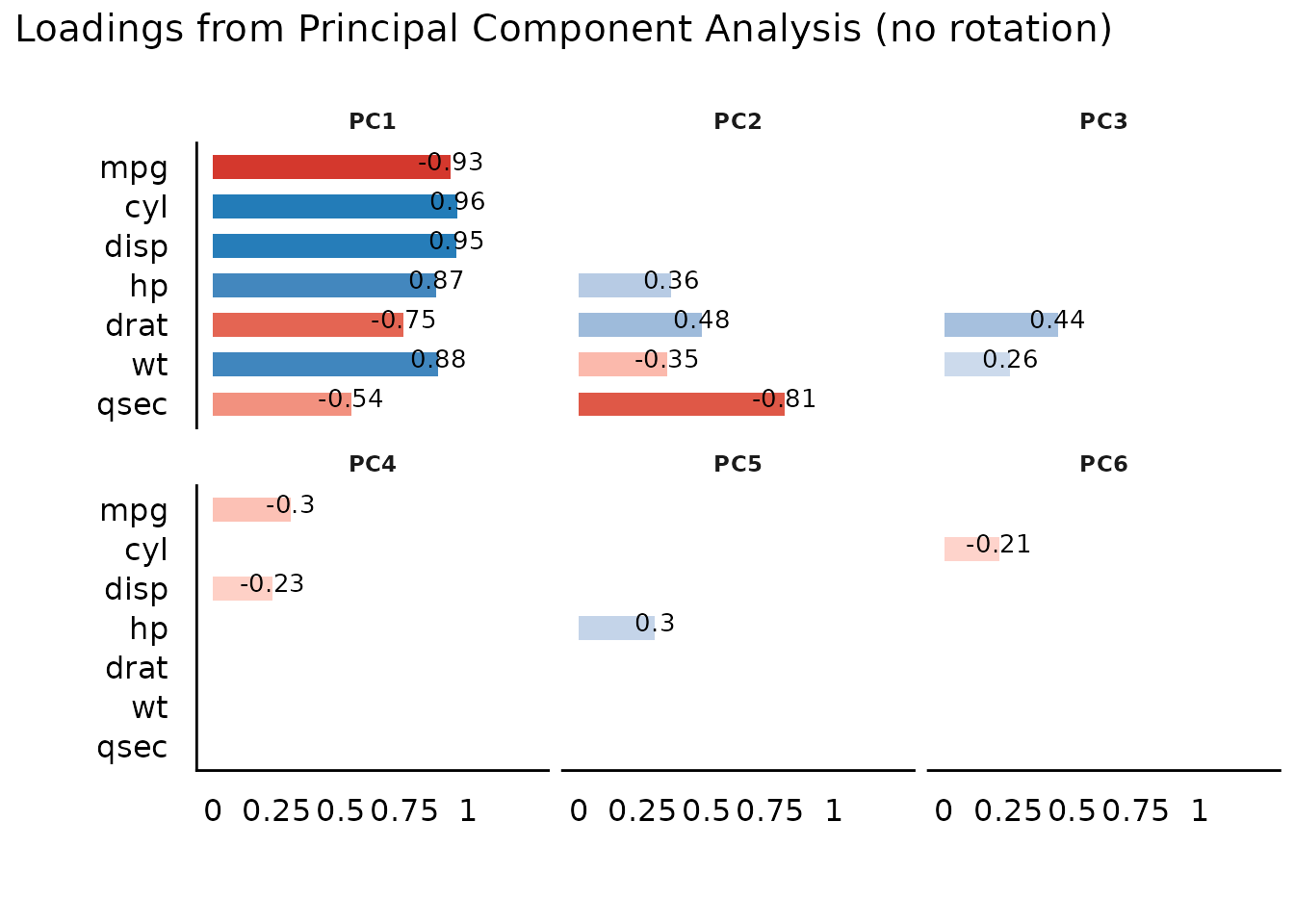plot(result, type = "line")## Number of Clusters to Retain (related function documentation) data(iris) result <- n_clusters(standardize(iris[, 1:4])) result #> # Method Agreement Procedure: #> #> The choice of 2 clusters is supported by 15 (51.72%) methods out of 29 (Elbow, Silhouette, Gap_Maechler2012, Gap_Dudoit2002, Ch, DB, Duda, Pseudot2, Beale, Ratkowsky, PtBiserial, Mcclain, Dunn, SDindex, Mixture (VVV)). plot(result)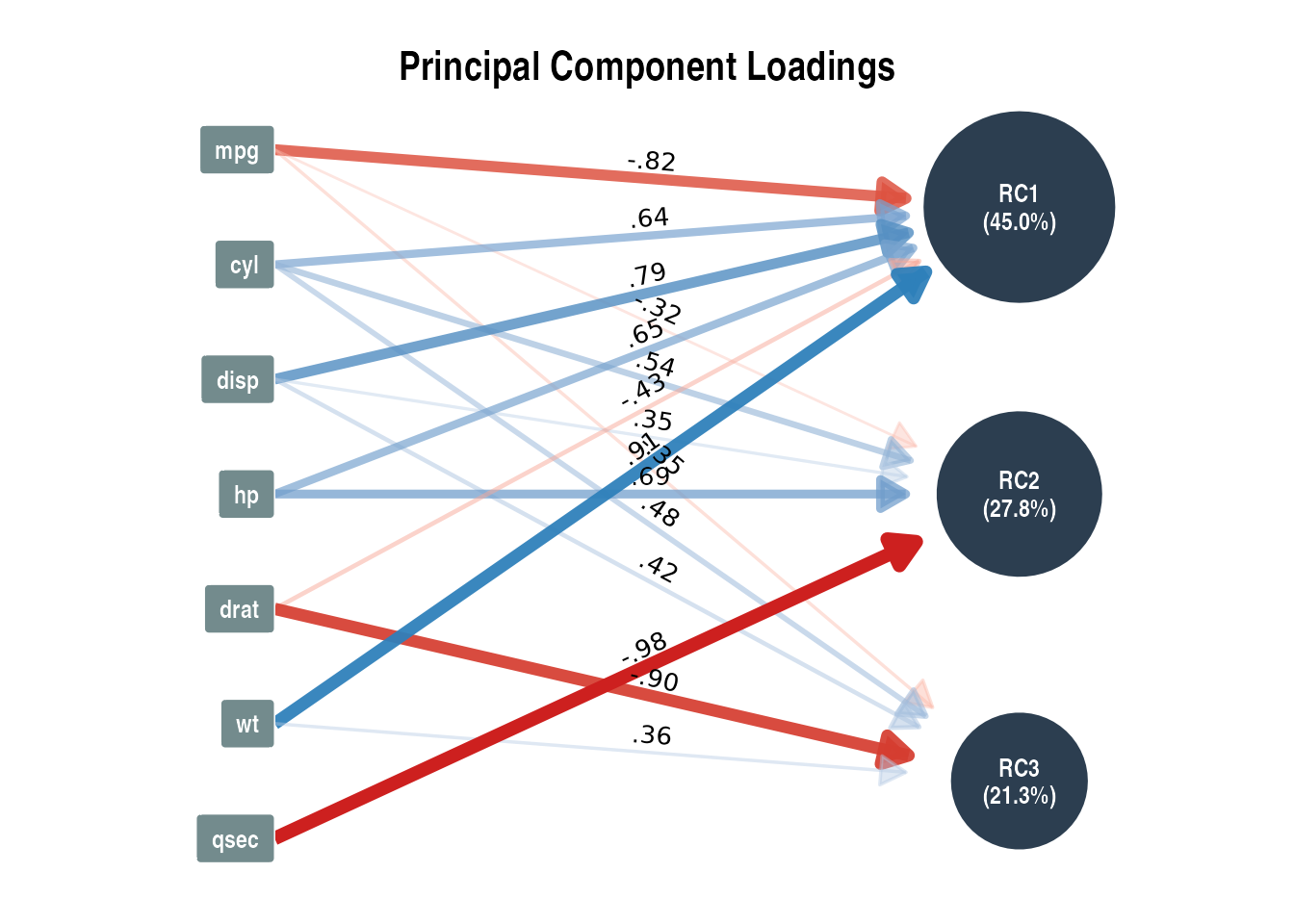plot(result, type = "line")## Description of Variable Distributions (related function documentation) ### Histogram for Numbers with Fractional Part data(iris) result <- describe_distribution(iris$Sepal.Length)
result
#> Mean |   SD |  IQR |        Range | Skewness | Kurtosis |   n | n_Missing
#> -------------------------------------------------------------------------
#> 5.84 | 0.83 | 1.30 | [4.30, 7.90] |     0.31 |    -0.55 | 150 |         0

plot(result)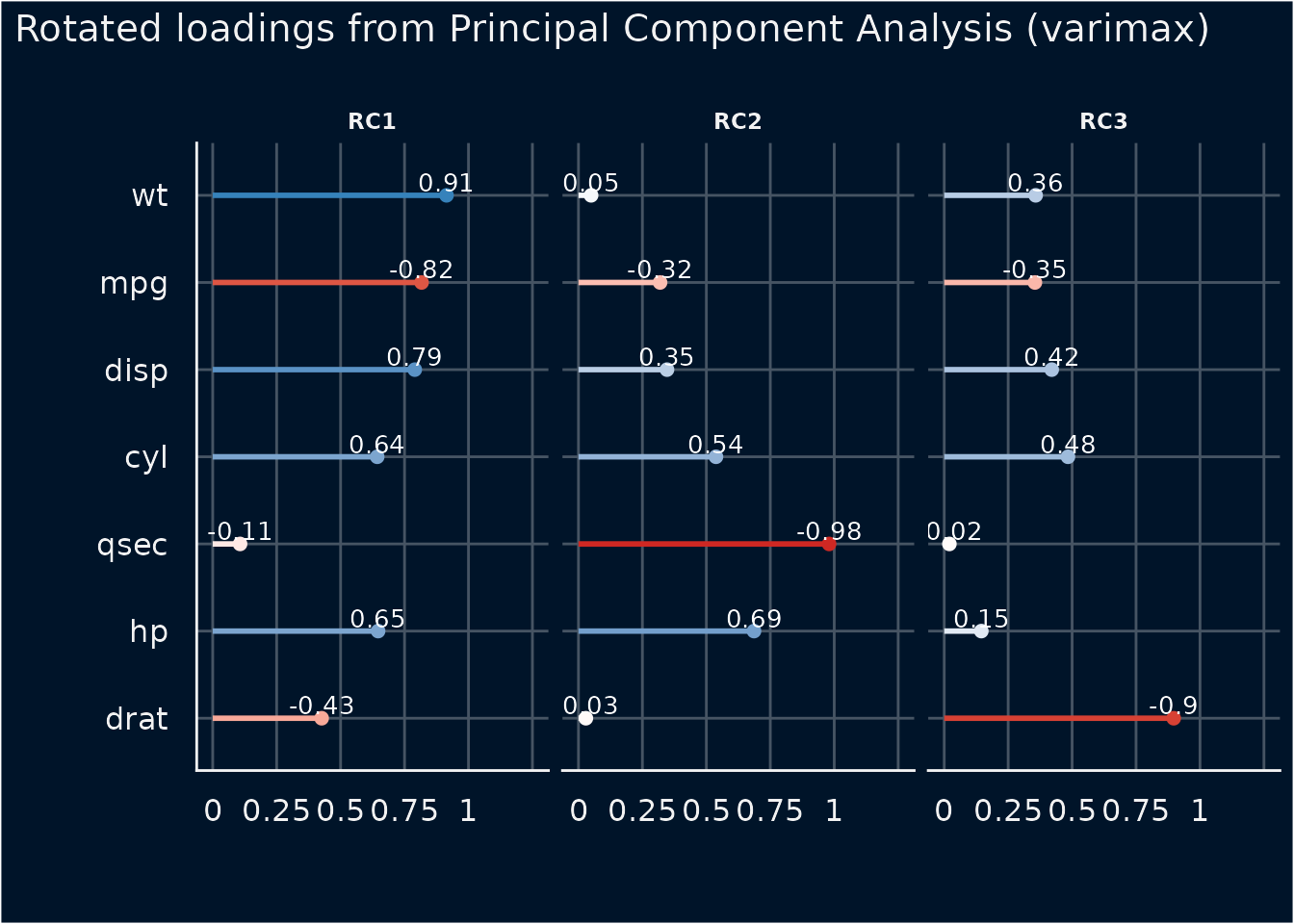plot(result, dispersion = TRUE)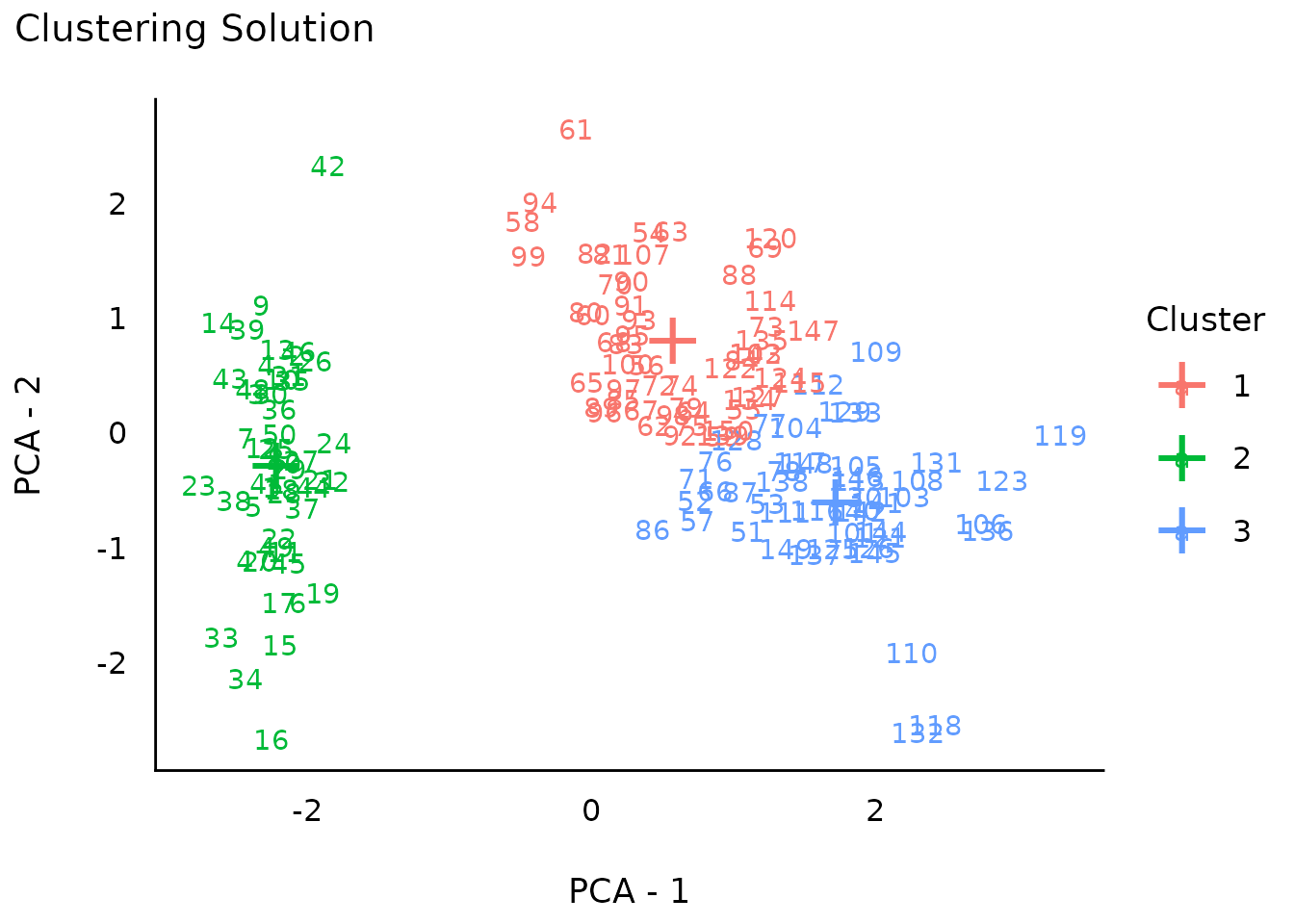### Thin Bars for Integer Values

set.seed(333)
x <- sample(1:100, 1000, replace = TRUE)
result <- describe_distribution(x)
result
#>  Mean |    SD |   IQR |          Range | Skewness | Kurtosis |    n | n_Missing
#> -------------------------------------------------------------------------------
#> 50.18 | 28.66 | 48.75 | [1.00, 100.00] |     0.02 |    -1.16 | 1000 |         0

plot(result)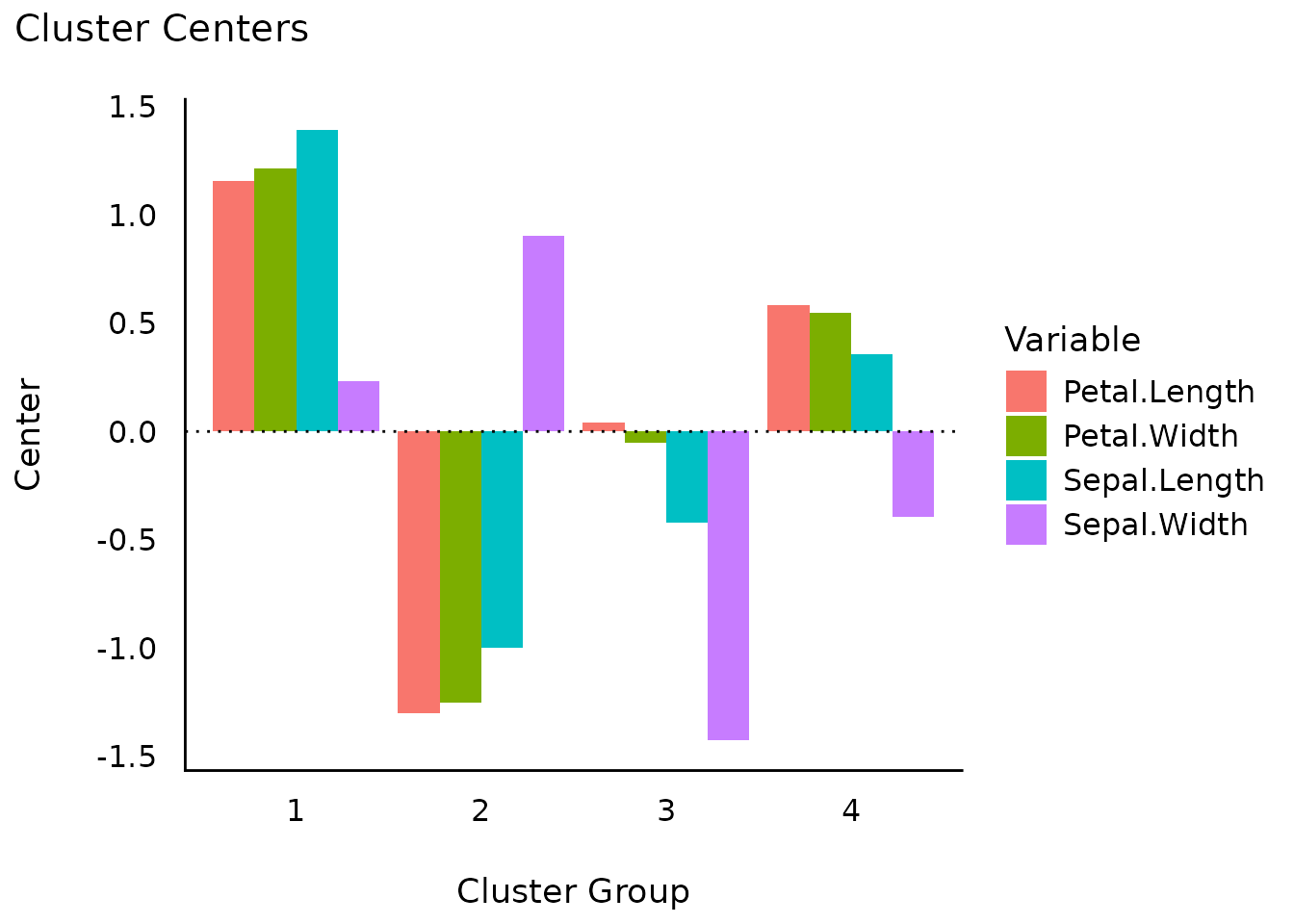### Use a Normal Curve instead of Ribbon

plot(result, dispersion = TRUE, dispersion_style = "curve")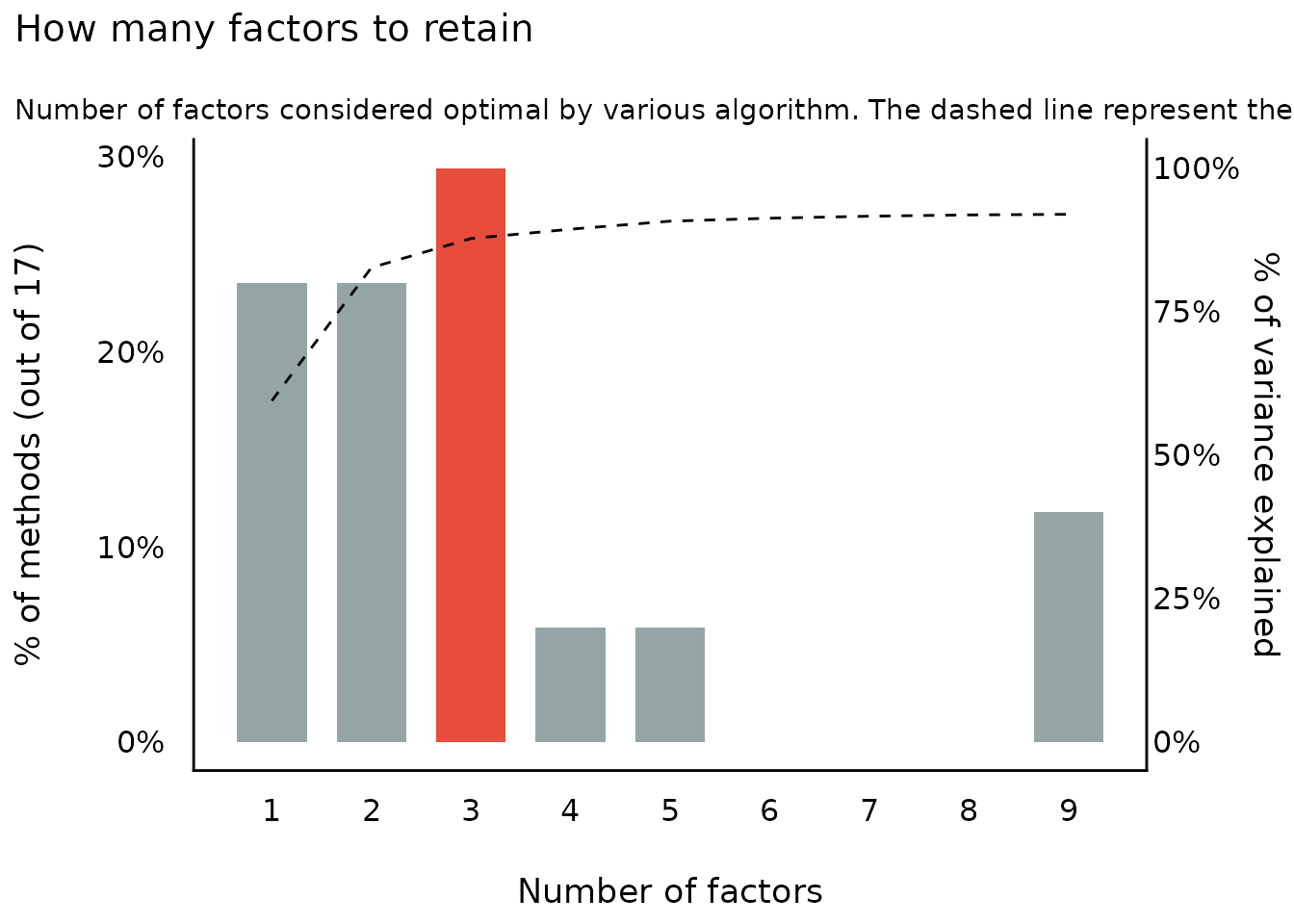### Highlighting Categories

set.seed(123)
result <- describe_distribution(sample(LETTERS[1:10], 1000, TRUE))

# highlight one category
plot(result, highlight = "D")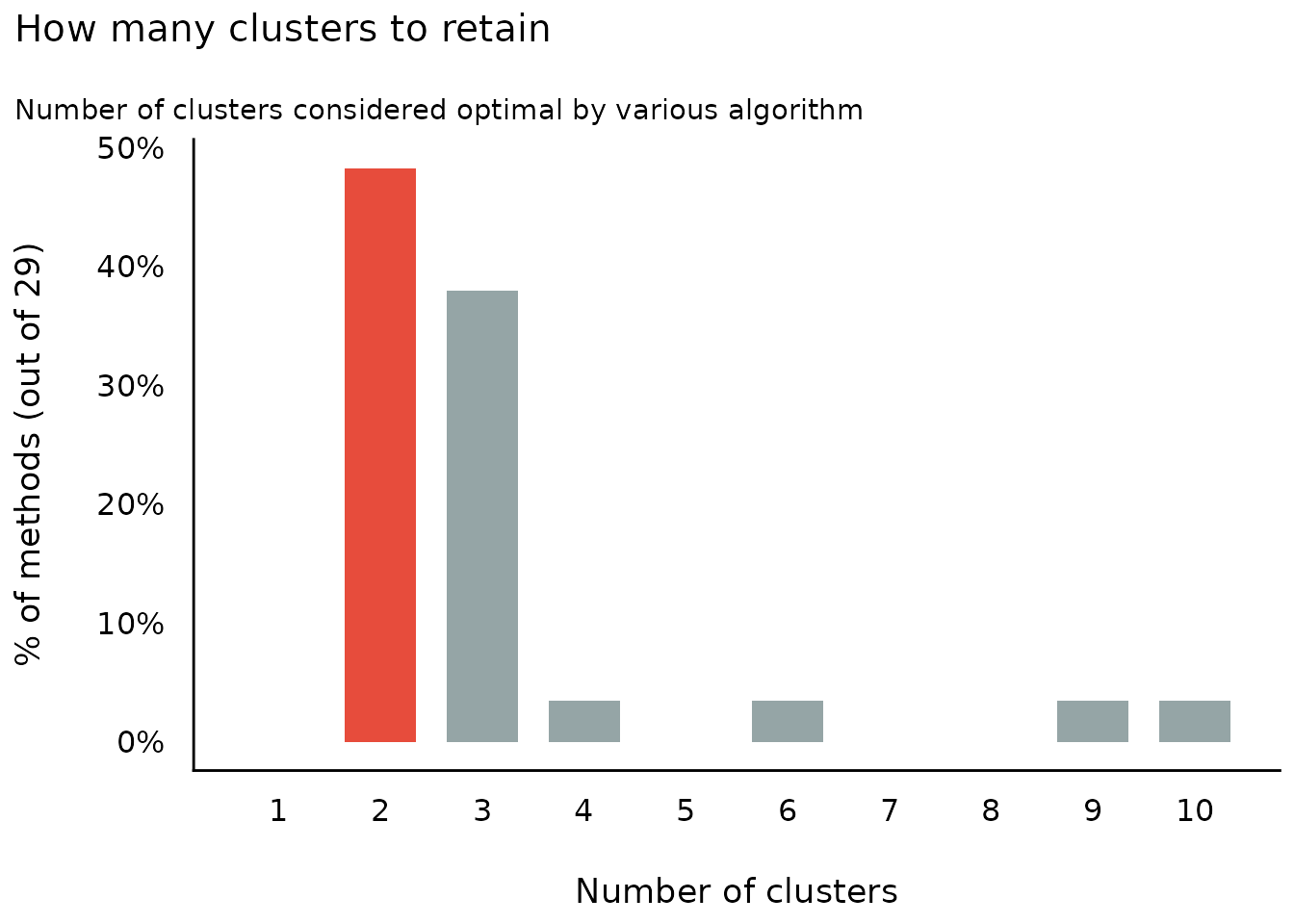# highlight multiple categories
plot(result, highlight = c("D", "H"), size_bar = .4)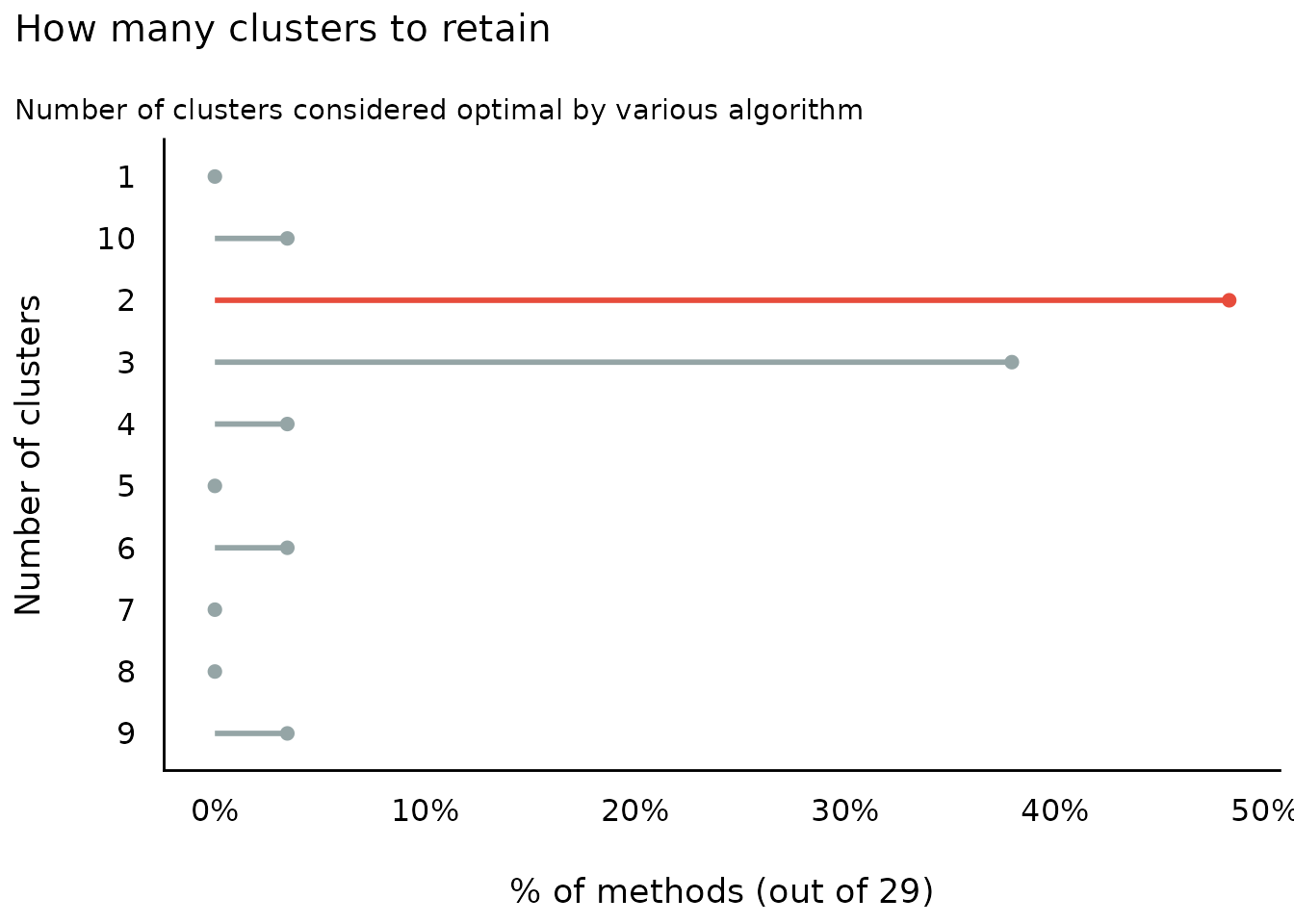# own color scales - pass a named vector to 'scale_fill_manual()'
# the name of the non-highlighted color is "no_highlight".
plot(result, highlight = c("D", "H", "A"), size_bar = .4) +
scale_fill_manual(values = c(D = "red", H = "green", A = "gold", no_highlight = "steelblue"))